### Dragon Moon Knew It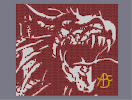Hover over the thumbnail for a full-size version.

Author ]{NO3 author:]{no3 bitesized dragon n-art nonplayable rated 2008-03-01 2008-03-27 5 by 139 people. \$Dragon Moon Knew It#]{NO3#none#11111111111111111111111111111111111111111111111111111111111111111111100000000000000000000000000000000000000000000000000000000000000000000000000000000000000000000000000000000000000000000000000000000000000000000000000000000000000000000000000000000000000000000000000000000000000000000000000000000000000000000000000000000000000000000000000000000000000000000000000000000000000000000000000000000000000000000000000000000000000000000000000000000000000000000000000000000000000000000000000000000000000000000000000000000000000000000000000000000000000000000000000000000000000000000000000000000000000000000000000000000000000000000000000000000000000000000000OOOOOOOOOOOOOOOOOOOOOOO1111111111111111111111111111111111111111111111|12^151,26!12^153,28!12^155,30!12^158,33!12^160,35!12^162,39!12^162,38!12^165,41!12^168,44!12^170,47!12^173,50!12^175,53!12^178,55!12^181,58!12^183,60!12^186,63!12^189,65!12^192,69!12^195,71!12^198,75!12^203,77!12^201,77!12^207,80!12^210,82!12^215,85!12^218,88!12^222,90!12^227,92!12^231,93!12^234,95!12^239,97!12^243,99!12^248,101!12^252,101!12^257,102!12^259,106!12^262,106!12^265,106!12^269,106!12^273,106!12^273,105!12^273,103!12^274,109!12^277,111!12^280,111!12^282,111!12^285,114!12^290,115!12^293,113!12^297,113!12^299,112!12^302,112!12^304,112!12^168,25!12^170,26!12^173,29!12^177,30!12^180,31!12^183,33!12^185,35!12^187,37!12^190,38!12^191,41!12^193,42!12^196,44!12^198,45!12^200,46!12^202,48!12^204,50!12^206,51!12^209,53!12^213,55!12^214,58!12^218,60!12^221,62!12^223,64!12^225,65!12^228,67!12^230,67!12^232,69!12^235,70!12^238,72!12^240,74!12^245,75!12^249,75!12^253,75!12^256,75!12^258,76!12^261,78!12^263,80!12^266,81!12^268,83!12^271,85!12^274,85!12^277,87!12^279,89!12^282,90!12^286,90!12^289,91!12^293,93!12^297,94!12^291,92!12^302,94!12^305,95!12^308,95!12^313,95!12^311,95!12^317,95!12^322,95!12^321,95!12^326,95!12^330,95!12^334,95!12^337,95!12^656,-15!12^337,101!12^337,105!12^340,94!12^344,91!12^348,88!12^353,86!12^358,84!12^364,81!12^360,76!12^356,71!12^351,69!12^347,67!12^347,67!12^341,64!12^336,63!12^331,63!12^325,62!12^321,62!12^315,62!12^311,62!12^306,62!12^301,62!12^296,62!12^291,59!12^286,58!12^283,55!12^285,48!12^291,47!12^296,46!12^301,46!12^307,46!12^311,48!12^315,49!12^323,49!12^321,49!12^329,50!12^335,50!12^339,52!12^344,55!12^348,55!12^352,57!12^356,58!12^359,60!12^363,62!12^365,65!12^369,68!12^372,71!12^373,71!12^378,75!12^383,74!12^390,76!12^396,75!12^404,76!12^403,80!12^400,82!12^395,85!12^389,87!12^379,86!12^384,89!12^380,90!12^378,94!12^373,94!12^373,93!12^369,96!12^365,98!12^362,100!12^359,101!12^355,102!12^354,103!12^351,105!12^349,105!12^345,107!12^341,107!12^334,112!12^330,112!12^329,113!12^323,115!12^320,117!12^319,120!12^307,114!12^307,119!12^309,123!12^313,126!12^317,122!12^408,76!12^404,74!12^403,70!12^400,67!12^398,64!12^397,59!12^393,54!12^396,50!12^400,48!12^405,49!12^407,55!12^410,60!12^412,64!12^414,67!12^418,69!12^421,69!12^421,74!12^421,79!12^416,79!12^409,79!12^411,79!12^425,65!12^421,62!12^419,58!12^418,53!12^422,60!12^418,49!12^417,45!12^422,41!12^420,41!12^428,41!12^431,41!12^432,44!12^433,47!12^433,50!12^435,54!12^429,45!12^432,51!12^434,55!12^438,59!12^439,60!12^442,61!12^442,63!12^444,67!12^446,68!12^448,70!12^427,69!12^430,72!12^433,75!12^435,77!12^436,82!12^438,84!12^440,86!12^443,88!12^446,90!12^450,91!12^454,94!12^453,72!12^457,73!12^457,69!12^457,66!12^457,61!12^457,57!12^457,54!12^457,52!12^457,47!12^460,45!12^464,45!12^469,48!12^470,54!12^471,57!12^471,61!12^471,65!12^472,67!12^473,70!12^474,72!12^475,75!12^476,79!12^477,81!12^477,84!12^485,88!12^482,87!12^490,91!12^494,94!12^498,94!12^503,92!12^506,94!12^511,92!12^515,90!12^518,90!12^521,89!12^524,89!12^528,89!12^502,94!12^533,88!12^535,88!12^539,88!12^543,88!12^547,85!12^550,85!12^554,85!12^558,88!12^557,91!12^555,94!12^554,95!12^560,89!12^564,87!12^567,85!12^571,85!12^577,85!12^581,84!12^585,84!12^588,87!12^587,91!12^585,92!12^588,92!12^590,90!12^593,91!12^599,93!12^600,92!12^596,87!12^598,88!12^596,84!12^595,80!12^594,77!12^592,74!12^591,70!12^590,66!12^590,62!12^594,61!12^598,61!12^602,65!12^604,68!12^606,73!12^607,77!12^608,81!12^609,86!12^610,90!12^611,93!12^612,96!12^613,100!12^614,103!12^615,106!12^618,109!12^623,111!12^627,116!12^623,112!12^630,119!12^634,119!12^637,119!12^639,119!12^641,119!12^647,119!12^645,119!12^650,119!12^654,121!12^658,121!12^662,123!12^665,127!12^669,130!12^670,132!12^673,135!12^678,135!12^680,138!12^684,140!12^686,143!12^687,147!12^455,99!12^455,104!12^457,110!12^460,113!12^465,114!12^468,116!12^472,119!12^473,122!12^471,123!12^468,124!12^465,126!12^459,126!12^454,127!12^448,130!12^447,134!12^448,139!12^452,141!12^456,142!12^459,142!12^462,142!12^467,143!12^472,144!12^475,147!12^481,149!12^485,148!12^489,148!12^495,147!12^498,147!12^502,144!12^506,141!12^510,142!12^514,143!12^519,144!12^521,143!12^524,139!12^528,136!12^535,133!12^539,131!12^542,128!12^545,125!12^580,94!12^584,94!12^592,93!12^596,93!12^549,122!12^553,119!12^557,116!12^562,113!12^567,111!12^572,109!12^575,109!12^577,109!12^581,109!12^586,109!12^590,109!12^594,111!12^598,114!12^602,118!12^605,121!12^607,124!12^610,127!12^612,132!12^615,138!12^617,140!12^614,136!12^618,143!12^619,148!12^624,159!12^628,172!12^628,178!12^632,181!12^637,187!12^641,192!12^645,196!12^649,195!12^650,191!12^650,187!12^652,184!12^655,182!12^659,180!12^663,180!12^665,182!12^668,187!12^668,190!12^669,195!12^670,198!12^671,201!12^690,151!12^692,154!12^693,157!12^695,162!12^696,165!12^696,170!12^670,205!12^670,210!12^669,215!12^669,225!12^669,219!12^669,222!12^670,228!12^672,234!12^676,238!12^678,242!12^679,245!12^676,252!12^674,257!12^697,174!12^697,178!12^699,184!12^698,189!12^698,194!12^697,201!12^697,199!12^697,206!12^697,214!12^698,210!12^697,220!12^697,224!12^697,229!12^696,236!12^696,235!12^693,242!12^691,247!12^687,252!12^686,256!12^682,262!12^678,268!12^681,265!12^684,260!12^688,253!12^690,246!12^668,275!12^673,272!12^675,270!12^671,262!12^667,265!12^665,267!12^667,260!12^667,257!12^667,253!12^667,250!12^667,246!12^667,243!12^665,240!12^662,235!12^659,232!12^657,230!12^654,230!12^652,230!12^649,230!12^648,232!12^665,278!12^665,283!12^663,289!12^662,294!12^664,286!12^698,182!12^532,135!12^509,92!12^660,299!12^658,303!12^655,307!12^651,311!12^649,314!12^646,316!12^640,314!12^640,309!12^641,306!12^643,301!12^642,305!12^644,298!12^645,292!12^646,286!12^646,284!12^644,273!12^644,266!12^643,269!12^643,261!12^677,248!12^640,262!12^639,264!12^635,268!12^643,288!12^642,289!12^641,275!12^638,277!12^633,281!12^631,283!12^628,286!12^624,290!12^620,294!12^616,298!12^612,302!12^607,306!12^605,309!12^601,312!12^597,314!12^592,316!12^589,319!12^587,321!12^586,325!12^584,326!12^581,329!12^579,332!12^577,336!12^574,339!12^573,344!12^639,292!12^636,295!12^632,298!12^628,301!12^625,304!12^621,307!12^617,311!12^614,314!12^610,317!12^605,320!12^600,324!12^597,326!12^594,330!12^591,334!12^589,337!12^586,341!12^584,345!12^604,321!12^609,304!12^635,279!12^641,317!12^571,349!12^570,355!12^570,351!12^582,350!12^582,354!12^582,356!12^585,354!12^589,351!12^592,351!12^567,358!12^562,362!12^563,362!12^558,364!12^555,368!12^551,370!12^546,372!12^540,372!12^536,372!12^531,373!12^527,373!12^522,373!12^517,373!12^513,369!12^512,365!12^510,360!12^507,356!12^505,352!12^502,349!12^498,348!12^497,344!12^497,340!12^493,339!12^489,339!12^486,343!12^486,346!12^486,353!12^485,350!12^489,357!12^485,360!12^488,360!12^484,363!12^482,365!12^487,366!12^490,366!12^481,368!12^475,370!12^695,239!12^541,389!12^546,388!12^553,386!12^558,382!12^563,380!12^567,380!12^571,382!12^578,382!12^582,378!12^584,375!12^588,373!12^590,372!12^574,383!12^558,382!12^553,385!12^549,389!12^539,390!12^533,391!12^530,391!12^528,390!12^523,390!12^517,389!12^513,389!12^509,388!12^506,388!12^503,388!12^498,387!12^492,387!12^488,385!12^483,384!12^478,384!12^473,386!12^467,387!12^463,387!12^478,369!12^470,372!12^468,372!12^464,373!12^460,373!12^458,373!12^456,374!12^451,376!12^449,374!12^445,375!12^441,378!12^452,374!12^435,383!12^435,386!12^458,388!12^453,388!12^448,388!12^444,388!12^440,388!12^437,388!12^397,120!12^402,120!12^408,120!12^408,120!12^414,120!12^420,120!12^426,120!12^430,123!12^433,127!12^429,131!12^423,132!12^419,137!12^418,142!12^413,146!12^406,147!12^402,147!12^411,147!12^417,144!12^422,133!12^428,133!12^402,132!12^402,138!12^402,144!12^398,147!12^394,147!12^389,147!12^398,144!12^383,141!12^383,144!12^385,145!12^391,120!12^389,120!12^386,122!12^381,124!12^377,127!12^374,131!12^373,135!12^371,139!12^370,143!12^370,146!12^371,150!12^374,154!12^378,157!12^384,159!12^389,159!12^391,156!12^391,152!12^388,149!12^415,143!12^417,140!12^418,136!12^419,132!12^419,65!12^416,63!12^414,58!12^410,55!12^409,50!12^413,49!12^414,53!12^342,78!12^348,78!12^348,84!12^342,84!12^348,72!12^342,72!12^336,72!12^336,78!12^336,84!12^324,78!12^330,78!12^330,84!12^324,84!12^330,72!12^324,72!12^318,72!12^318,78!12^318,84!12^306,78!12^312,78!12^312,84!12^306,84!12^312,72!12^306,72!12^300,72!12^300,78!12^300,84!12^288,72!12^294,72!12^294,78!12^288,78!12^294,66!12^288,66!12^282,66!12^282,72!12^282,78!12^270,66!12^276,66!12^276,72!12^270,72!12^276,60!12^270,60!12^264,60!12^264,66!12^264,72!12^270,48!12^276,48!12^276,54!12^270,54!12^276,42!12^270,42!12^264,42!12^264,48!12^264,54!12^270,30!12^276,30!12^276,36!12^270,36!12^276,24!12^270,24!12^264,24!12^264,30!12^264,36!12^252,30!12^258,30!12^258,36!12^252,36!12^258,24!12^252,24!12^246,24!12^246,30!12^246,36!12^252,48!12^258,48!12^258,54!12^252,54!12^258,42!12^252,42!12^246,42!12^246,48!12^246,54!12^252,66!12^258,66!12^258,72!12^252,72!12^258,60!12^252,60!12^246,60!12^246,66!12^246,72!12^234,30!12^240,30!12^240,36!12^234,36!12^240,24!12^234,24!12^228,24!12^228,30!12^228,36!12^234,48!12^240,48!12^240,54!12^234,54!12^240,42!12^234,42!12^228,42!12^228,48!12^228,54!12^216,30!12^222,30!12^222,36!12^216,36!12^222,24!12^216,24!12^210,24!12^210,30!12^210,36!12^198,30!12^204,30!12^204,36!12^198,36!12^204,24!12^198,24!12^192,24!12^192,30!12^192,36!12^216,48!12^222,48!12^222,54!12^216,54!12^222,42!12^216,42!12^210,42!12^210,48!12^210,54!12^288,30!12^294,30!12^294,36!12^288,36!12^294,24!12^288,24!12^282,24!12^282,30!12^282,36!12^306,30!12^312,30!12^312,36!12^306,36!12^312,24!12^306,24!12^300,24!12^300,30!12^300,36!12^324,30!12^330,30!12^330,36!12^324,36!12^330,24!12^324,24!12^318,24!12^318,30!12^318,36!12^354,80!12^360,78!12^354,78!12^354,72!12^342,66!12^336,66!12^330,66!12^324,66!12^318,66!12^312,66!12^306,66!12^300,66!12^288,60!12^282,60!12^282,54!12^282,48!12^282,42!12^288,42!12^294,42!12^300,42!12^306,42!12^312,42!12^318,42!12^324,42!12^330,42!12^330,48!12^318,48!12^342,90!12^336,90!12^324,90!12^330,90!12^318,90!12^312,90!12^306,90!12^300,90!12^294,84!12^294,90!12^288,84!12^282,84!12^276,78!12^276,84!12^270,78!12^264,78!12^240,66!12^240,72!12^240,60!12^234,60!12^228,60!12^222,60!12^234,66!12^204,42!12^204,48!12^198,42!12^186,24!12^186,30!12^180,24!12^174,24!12^642,233!12^644,233!12^638,231!12^636,228!12^636,225!12^630,270!12^629,271!12^625,274!12^623,276!12^619,279!12^616,282!12^613,282!12^613,277!12^610,279!12^606,277!12^604,278!12^573,238!12^573,233!12^573,229!12^573,241!12^571,249!12^571,254!12^570,256!12^571,248!12^571,255!12^568,260!12^568,262!12^567,264!12^566,266!12^565,269!12^564,272!12^563,274!12^562,278!12^561,280!12^559,284!12^558,286!12^557,289!12^555,291!12^577,222!12^573,224!12^573,219!12^573,214!12^571,210!12^569,208!12^566,207!12^565,205!12^562,205!12^559,205!12^555,205!12^552,206!12^550,207!12^550,212!12^549,214!12^549,221!12^549,218!12^549,221!12^550,224!12^550,227!12^550,232!12^550,235!12^550,239!12^550,243!12^550,245!12^553,293!12^549,252!12^549,249!12^549,258!12^548,262!12^549,264!12^548,269!12^548,273!12^548,276!12^547,279!12^547,283!12^547,286!12^547,290!12^546,291!12^547,294!12^634,234!12^634,238!12^634,242!12^633,246!12^633,249!12^632,252!12^632,254!12^630,258!12^629,262!12^627,264!12^626,269!12^625,273!12^623,275!12^613,273!12^613,268!12^613,265!12^613,261!12^613,259!12^614,253!12^614,249!12^614,245!12^614,242!12^549,255!12^584,223!12^584,227!12^584,232!12^611,238!12^611,246!12^610,250!12^610,254!12^610,257!12^609,260!12^609,263!12^608,267!12^608,270!12^606,274!12^604,279!12^587,239!12^587,250!12^586,241!12^586,237!12^587,247!12^587,253!12^588,256!12^588,259!12^589,262!12^589,264!12^589,265!12^590,267!12^590,271!12^590,272!12^590,274!12^590,276!12^590,277!12^595,279!12^599,276!12^600,274!12^602,269!12^604,266!12^605,263!12^607,258!12^610,249!12^603,277!12^606,272!12^609,275!12^613,236!12^613,233!12^612,241!12^573,245!12^620,149!12^623,154!12^624,157!12^625,160!12^627,170!12^626,166!12^624,161!12^621,151!12^632,181!12^634,184!12^637,186!12^639,188!12^641,190!12^643,193!12^627,174!12^584,218!12^586,216!12^590,214!12^592,214!12^597,213!12^602,212!12^609,214!12^606,213!12^614,216!12^618,217!12^622,217!12^625,217!12^630,219!12^634,222!12^544,230!12^542,236!12^540,238!12^538,241!12^538,244!12^536,246!12^535,250!12^533,252!12^532,255!12^529,258!12^528,263!12^527,265!12^526,269!12^523,270!12^521,268!12^519,264!12^518,260!12^518,256!12^516,254!12^516,250!12^516,246!12^544,227!12^540,227!12^534,229!12^530,232!12^525,233!12^523,237!12^520,239!12^518,241!12^540,228!12^530,253!12^532,249!12^533,245!12^535,242!12^496,248!12^499,248!12^502,248!12^507,247!12^507,251!12^505,256!12^503,261!12^502,265!12^499,269!12^498,273!12^501,269!12^490,249!12^490,254!12^490,258!12^490,264!12^490,268!12^490,273!12^494,276!12^496,274!12^510,244!12^516,243!12^504,245!12^509,243!12^513,240!12^515,239!12^521,234!12^524,231!12^525,230!12^526,228!12^526,225!12^525,220!12^525,219!12^530,226!12^530,224!12^531,223!12^532,222!12^535,217!12^536,214!12^539,209!12^542,207!12^546,204!12^547,205!12^546,212!12^545,216!12^546,222!12^540,222!12^534,222!12^540,217!12^542,211!12^545,226!12^549,201!12^552,201!12^558,203!12^563,203!12^567,200!12^570,200!12^575,199!12^577,199!12^579,199!12^582,199!12^585,199!12^589,199!12^592,199!12^597,199!12^600,199!12^603,199!12^606,197!12^607,194!12^609,192!12^609,191!12^610,187!12^611,183!12^486,248!12^484,248!12^480,248!12^479,244!12^480,240!12^484,237!12^485,233!12^487,230!12^487,229!12^490,226!12^492,223!12^494,220!12^496,218!12^497,214!12^499,212!12^501,210!12^503,209!12^507,209!12^511,209!12^513,208!12^508,214!12^506,205!12^506,201!12^510,197!12^513,194!12^516,190!12^520,189!12^525,186!12^527,183!12^532,182!12^536,186!12^536,187!12^541,181!12^545,179!12^552,176!12^545,179!12^547,178!12^554,172!12^559,170!12^563,170!12^569,170!12^576,172!12^579,172!12^583,172!12^587,172!12^590,174!12^595,174!12^600,176!12^604,177!12^608,177!12^610,178!12^600,175!12^575,172!12^568,171!12^538,184!12^537,184!12^549,177!12^614,176!12^618,174!12^620,170!12^620,167!12^620,162!12^618,157!12^617,155!12^615,153!12^613,152!12^611,150!12^609,148!12^607,145!12^605,143!12^601,141!12^597,139!12^594,138!12^589,137!12^586,137!12^605,155!12^582,138!12^577,138!12^573,138!12^568,138!12^564,140!12^563,144!12^561,147!12^558,149!12^587,170!12^583,169!12^580,168!12^576,167!12^572,167!12^567,166!12^564,164!12^563,164!12^561,163!12^557,159!12^557,155!12^555,157!12^553,159!12^551,161!12^548,163!12^543,163!12^540,162!12^541,157!12^537,156!12^535,153!12^535,149!12^536,147!12^541,145!12^544,143!12^547,142!12^549,142!12^553,141!12^556,141!12^558,143!12^560,140!12^539,144!12^536,192!12^593,194!12^593,192!12^574,177!12^578,177!12^519,210!12^524,216!12^342,30!12^348,30!12^348,36!12^342,36!12^348,24!12^342,24!12^336,24!12^336,30!12^336,36!12^360,30!12^366,30!12^366,36!12^360,36!12^366,24!12^360,24!12^354,24!12^354,30!12^354,36!12^378,30!12^384,30!12^384,36!12^378,36!12^384,24!12^378,24!12^372,24!12^372,30!12^372,36!12^396,30!12^402,30!12^402,36!12^396,36!12^402,24!12^396,24!12^390,24!12^390,30!12^390,36!12^414,30!12^420,30!12^420,36!12^414,36!12^420,24!12^414,24!12^408,24!12^408,30!12^408,36!12^432,30!12^438,30!12^438,36!12^432,36!12^438,24!12^432,24!12^426,24!12^426,30!12^426,36!12^450,30!12^456,30!12^456,36!12^450,36!12^456,24!12^450,24!12^444,24!12^444,30!12^444,36!12^384,48!12^390,48!12^390,54!12^384,54!12^390,42!12^384,42!12^378,42!12^378,48!12^378,54!12^366,48!12^372,48!12^372,54!12^366,54!12^372,42!12^366,42!12^360,42!12^360,48!12^360,54!12^390,66!12^396,66!12^396,72!12^390,72!12^396,60!12^390,60!12^384,60!12^384,66!12^384,72!12^444,48!12^450,48!12^450,54!12^444,54!12^450,42!12^444,42!12^438,42!12^438,48!12^438,54!12^468,30!12^474,30!12^474,36!12^468,36!12^474,24!12^468,24!12^462,24!12^462,30!12^462,36!12^486,30!12^492,30!12^492,36!12^486,36!12^492,24!12^486,24!12^480,24!12^480,30!12^480,36!12^486,48!12^492,48!12^492,54!12^486,54!12^492,42!12^486,42!12^480,42!12^480,48!12^480,54!12^486,66!12^492,66!12^492,72!12^486,72!12^492,60!12^486,60!12^480,60!12^480,66!12^480,72!12^504,66!12^510,66!12^510,72!12^504,72!12^510,60!12^504,60!12^498,60!12^498,66!12^498,72!12^504,48!12^510,48!12^510,54!12^504,54!12^510,42!12^504,42!12^498,42!12^498,48!12^498,54!12^504,30!12^510,30!12^510,36!12^504,36!12^510,24!12^504,24!12^498,24!12^498,30!12^498,36!12^522,30!12^528,30!12^528,36!12^522,36!12^528,24!12^522,24!12^516,24!12^516,30!12^516,36!12^522,48!12^528,48!12^528,54!12^522,54!12^528,42!12^522,42!12^516,42!12^516,48!12^516,54!12^522,66!12^528,66!12^528,72!12^522,72!12^528,60!12^522,60!12^516,60!12^516,66!12^516,72!12^498,84!12^504,84!12^504,90!12^498,90!12^504,78!12^498,78!12^492,78!12^492,84!12^492,90!12^540,30!12^546,30!12^546,36!12^540,36!12^546,24!12^540,24!12^534,24!12^534,30!12^534,36!12^558,30!12^564,30!12^564,36!12^558,36!12^564,24!12^558,24!12^552,24!12^552,30!12^552,36!12^378,72!12^378,66!12^378,60!12^372,60!12^366,60!12^372,66!12^354,48!12^354,54!12^348,48!12^342,48!12^336,48!12^336,42!12^342,42!12^348,42!12^354,42!12^390,42!12^396,42!12^396,48!12^402,42!12^408,42!12^414,42!12^450,60!12^450,66!12^444,60!12^456,42!12^468,42!12^462,42!12^474,42!12^474,48!12^474,54!12^475,58!12^477,62!12^477,66!12^486,78!12^480,78!12^486,84!12^481,82!12^593,369!12^597,367!12^601,365!12^608,364!12^612,362!12^619,361!12^623,360!12^630,359!12^637,359!12^641,359!12^647,359!12^651,359!12^659,360!12^663,361!12^668,364!12^674,367!12^680,371!12^685,374!12^689,376!12^693,377!12^695,378!12^699,380!12^702,381!12^705,382!12^684,375!12^682,373!12^679,370!12^678,369!12^670,364!12^655,360!12^599,351!12^596,351!12^605,351!12^608,351!12^613,350!12^615,350!12^620,350!12^623,348!12^627,347!12^628,347!12^632,347!12^637,347!12^643,347!12^644,347!12^649,347!12^652,347!12^656,347!12^659,347!12^663,347!12^668,348!12^671,349!12^674,350!12^678,352!12^680,353!12^682,355!12^685,356!12^687,358!12^691,360!12^694,360!12^697,362!12^700,362!12^702,363!12^558,390!12^558,396!12^552,390!12^552,396!12^552,408!12^552,402!12^552,414!12^552,420!12^552,426!12^552,432!12^552,438!12^552,444!12^552,450!12^552,456!12^552,462!12^552,468!12^552,474!12^552,480!12^552,486!12^555,488!12^558,487!12^562,483!12^566,481!12^567,478!12^571,474!12^576,472!12^580,472!12^581,478!12^580,485!12^580,482!12^578,488!12^575,494!12^577,493!12^573,498!12^570,500!12^569,503!12^568,506!12^568,510!12^568,513!12^568,517!12^566,520!12^565,524!12^564,529!12^563,533!12^563,536!12^562,538!12^560,540!12^557,543!12^554,546!12^551,548!12^549,553!12^544,555!12^541,556!12^536,557!12^531,557!12^528,556!12^524,555!12^520,553!12^516,553!12^510,553!12^508,555!12^505,557!12^503,557!12^502,557!12^501,556!12^500,551!12^498,550!12^494,548!12^490,548!12^486,548!12^480,546!12^476,545!12^473,544!12^469,537!12^471,543!12^469,538!12^466,534!12^465,532!12^464,529!12^462,526!12^461,522!12^460,519!12^459,518!12^461,515!12^457,513!12^457,510!12^456,507!12^456,504!12^455,498!12^455,494!12^452,493!12^447,493!12^443,492!12^438,490!12^440,491!12^435,495!12^431,495!12^426,495!12^420,492!12^417,490!12^415,488!12^413,484!12^409,481!12^406,478!12^403,474!12^403,473!12^397,471!12^395,468!12^392,465!12^391,463!12^389,460!12^389,457!12^391,457!12^393,456!12^398,456!12^403,459!12^399,453!12^396,449!12^392,446!12^390,444!12^388,440!12^386,436!12^382,433!12^381,430!12^379,428!12^540,48!12^546,48!12^546,54!12^540,54!12^546,42!12^540,42!12^534,42!12^534,48!12^534,54!12^558,48!12^564,48!12^564,54!12^558,54!12^564,42!12^558,42!12^552,42!12^552,48!12^552,54!12^540,66!12^546,66!12^546,72!12^540,72!12^546,60!12^540,60!12^534,60!12^534,66!12^534,72!12^558,66!12^564,66!12^564,72!12^558,72!12^564,60!12^558,60!12^552,60!12^552,66!12^552,72!12^576,66!12^582,66!12^582,72!12^576,72!12^582,60!12^576,60!12^570,60!12^570,66!12^570,72!12^576,48!12^582,48!12^582,54!12^576,54!12^582,42!12^576,42!12^570,42!12^570,48!12^570,54!12^576,30!12^582,30!12^582,36!12^576,36!12^582,24!12^576,24!12^570,24!12^570,30!12^570,36!12^594,30!12^600,30!12^600,36!12^594,36!12^600,24!12^594,24!12^588,24!12^588,30!12^588,36!12^612,30!12^618,30!12^618,36!12^612,36!12^618,24!12^612,24!12^606,24!12^606,30!12^606,36!12^630,30!12^636,30!12^636,36!12^630,36!12^636,24!12^630,24!12^624,24!12^624,30!12^624,36!12^594,48!12^600,48!12^600,54!12^594,54!12^600,42!12^594,42!12^588,42!12^588,48!12^588,54!12^612,48!12^618,48!12^618,54!12^612,54!12^618,42!12^612,42!12^606,42!12^606,48!12^606,54!12^630,48!12^636,48!12^636,54!12^630,54!12^636,42!12^630,42!12^624,42!12^624,48!12^624,54!12^630,66!12^636,66!12^636,72!12^630,72!12^636,60!12^630,60!12^624,60!12^624,66!12^624,72!12^630,84!12^636,84!12^636,90!12^630,90!12^636,78!12^630,78!12^624,78!12^624,84!12^624,90!12^630,102!12^636,102!12^636,108!12^630,108!12^636,96!12^630,96!12^624,96!12^624,102!12^624,108!12^648,102!12^654,102!12^654,108!12^648,108!12^654,96!12^648,96!12^642,96!12^642,102!12^642,108!12^648,84!12^654,84!12^654,90!12^648,90!12^654,78!12^648,78!12^642,78!12^642,84!12^642,90!12^648,66!12^654,66!12^654,72!12^648,72!12^654,60!12^648,60!12^642,60!12^642,66!12^642,72!12^648,48!12^654,48!12^654,54!12^648,54!12^654,42!12^648,42!12^642,42!12^642,48!12^642,54!12^648,30!12^654,30!12^654,36!12^648,36!12^654,24!12^648,24!12^642,24!12^642,30!12^642,36!12^666,30!12^672,30!12^672,36!12^666,36!12^672,24!12^666,24!12^660,24!12^660,30!12^660,36!12^666,48!12^672,48!12^672,54!12^666,54!12^672,42!12^666,42!12^660,42!12^660,48!12^660,54!12^666,66!12^672,66!12^672,72!12^666,72!12^672,60!12^666,60!12^660,60!12^660,66!12^660,72!12^666,84!12^672,84!12^672,90!12^666,90!12^672,78!12^666,78!12^660,78!12^660,84!12^660,90!12^666,102!12^672,102!12^672,108!12^666,108!12^672,96!12^666,96!12^660,96!12^660,102!12^660,108!12^684,102!12^690,102!12^690,108!12^684,108!12^690,96!12^684,96!12^678,96!12^678,102!12^678,108!12^684,84!12^690,84!12^690,90!12^684,90!12^690,78!12^684,78!12^678,78!12^678,84!12^678,90!12^684,66!12^690,66!12^690,72!12^684,72!12^690,60!12^684,60!12^678,60!12^678,66!12^678,72!12^684,48!12^690,48!12^690,54!12^684,54!12^690,42!12^684,42!12^678,42!12^678,48!12^678,54!12^684,30!12^690,30!12^690,36!12^684,36!12^690,24!12^684,24!12^678,24!12^678,30!12^678,36!12^684,120!12^690,120!12^690,126!12^684,126!12^690,114!12^684,114!12^678,114!12^678,120!12^678,126!12^510,78!12^510,84!12^510,90!12^516,84!12^528,84!12^522,84!12^522,78!12^528,78!12^516,78!12^534,78!12^534,84!12^540,84!12^540,78!12^546,78!12^546,84!12^552,78!12^558,78!12^558,84!12^564,84!12^564,78!12^570,78!12^576,78!12^573,84!12^582,78!12^588,78!12^590,84!12^593,86!12^588,72!12^588,66!12^588,60!12^594,60!12^600,60!12^606,60!12^606,66!12^607,70!12^612,60!12^618,60!12^618,66!12^612,66!12^612,72!12^618,72!12^618,78!12^612,78!12^612,84!12^618,84!12^618,90!12^612,90!12^618,96!12^618,102!12^621,105!12^630,114!12^636,114!12^642,114!12^648,114!12^654,114!12^660,114!12^666,114!12^672,114!12^672,120!12^666,120!12^660,120!12^672,126!12^666,126!12^672,132!12^678,132!12^684,132!12^690,132!12^690,138!12^684,138!12^690,144!12^580,90!12^481,474!12^484,470!12^484,465!12^487,460!12^494,462!12^495,465!12^496,470!12^496,473!12^496,478!12^497,480!12^500,483!12^504,485!12^507,486!12^510,490!12^512,494!12^512,498!12^516,501!12^518,503!12^523,505!12^523,509!12^523,513!12^522,517!12^525,516!12^528,514!12^531,513!12^532,511!12^535,511!12^533,507!12^532,505!12^531,503!12^530,502!12^529,499!12^525,495!12^525,492!12^523,489!12^520,486!12^520,481!12^519,476!12^519,473!12^519,467!12^521,466!12^520,463!12^519,459!12^518,455!12^515,451!12^512,448!12^511,445!12^515,458!12^514,458!12^512,458!12^510,458!12^506,457!12^502,456!12^497,456!12^493,455!12^491,455!12^488,453!12^484,450!12^482,447!12^479,446!12^476,443!12^474,439!12^470,436!12^475,442!12^477,449!12^477,452!12^479,456!12^480,460!12^480,464!12^479,471!12^480,471!12^477,464!12^481,467!12^479,458!12^485,456!12^483,459!12^481,452!12^492,458!12^497,461!12^503,463!12^503,462!12^510,462!12^516,462!12^516,468!12^510,468!12^504,468!12^498,468!12^498,474!12^510,474!12^504,474!12^516,474!12^516,480!12^510,480!12^504,480!12^516,486!12^510,486!12^515,492!12^520,492!12^517,497!12^521,500!12^523,498!12^526,500!12^527,504!12^528,508!12^526,509!12^547,396!12^546,395!12^542,394!12^537,394!12^536,399!12^535,403!12^531,405!12^527,406!12^523,406!12^522,403!12^518,399!12^517,395!12^513,393!12^510,393!12^507,393!12^505,393!12^525,396!12^523,396!12^520,395!12^524,402!12^527,402!12^528,399!12^529,397!12^531,396!12^531,401!12^529,412!12^529,420!12^529,417!12^534,418!12^533,422!12^529,427!12^526,428!12^521,431!12^526,429!12^527,425!12^525,421!12^525,417!12^521,419!12^514,436!12^509,438!12^507,436!12^512,437!12^517,434!12^498,392!12^492,392!12^502,392!12^495,391!12^490,391!12^487,390!12^484,390!12^479,390!12^474,390!12^486,392!12^476,392!12^473,392!12^469,392!12^465,392!12^461,392!12^457,391!12^501,433!12^497,430!12^498,410!12^494,410!12^496,406!12^496,403!12^496,398!12^501,411!12^491,411!12^490,414!12^490,417!12^492,420!12^494,422!12^495,424!12^497,425!12^495,430!12^491,424!12^492,427!12^497,429!12^502,432!12^501,430!12^488,423!12^488,421!12^487,417!12^486,413!12^486,411!12^487,409!12^490,403!12^493,398!12^492,404!12^488,406!12^484,411!12^484,413!12^482,416!12^483,420!12^508,528!12^508,531!12^503,534!12^505,536!12^505,539!12^508,540!12^513,541!12^517,541!12^521,541!12^525,541!12^526,541!12^530,540!12^527,538!12^525,535!12^523,532!12^520,531!12^520,535!12^515,535!12^511,535!12^508,524!12^510,531!12^486,524!12^484,522!12^483,519!12^482,516!12^471,494!12^469,494!12^452,473!12^452,471!12^452,469!12^451,464!12^452,477!12^452,480!12^534,360!12^540,360!12^540,366!12^534,366!12^540,354!12^534,354!12^528,354!12^528,360!12^528,366!12^534,342!12^540,342!12^540,348!12^534,348!12^540,336!12^534,336!12^528,336!12^528,342!12^528,348!12^552,342!12^558,342!12^558,348!12^552,348!12^558,336!12^552,336!12^546,336!12^546,342!12^546,348!12^552,324!12^558,324!12^558,330!12^552,330!12^558,318!12^552,318!12^546,318!12^546,324!12^546,330!12^534,324!12^540,324!12^540,330!12^534,330!12^540,318!12^534,318!12^528,318!12^528,324!12^528,330!12^534,306!12^540,306!12^540,312!12^534,312!12^540,300!12^534,300!12^528,300!12^528,306!12^528,312!12^552,306!12^558,306!12^558,312!12^552,312!12^558,300!12^552,300!12^546,300!12^546,306!12^546,312!12^570,306!12^576,306!12^576,312!12^570,312!12^576,300!12^570,300!12^564,300!12^564,306!12^564,312!12^588,306!12^594,306!12^594,312!12^588,312!12^594,300!12^588,300!12^582,300!12^582,306!12^582,312!12^570,288!12^576,288!12^576,294!12^570,294!12^576,282!12^570,282!12^564,282!12^564,288!12^564,294!12^588,288!12^594,288!12^594,294!12^588,294!12^594,282!12^588,282!12^582,282!12^582,288!12^582,294!12^570,324!12^576,324!12^576,330!12^570,330!12^576,318!12^570,318!12^564,318!12^564,324!12^564,330!12^516,306!12^522,306!12^522,312!12^516,312!12^522,300!12^516,300!12^510,300!12^510,306!12^510,312!12^516,324!12^522,324!12^522,330!12^516,330!12^522,318!12^516,318!12^510,318!12^510,324!12^510,330!12^516,342!12^522,342!12^522,348!12^516,348!12^522,336!12^516,336!12^510,336!12^510,342!12^510,348!12^498,306!12^504,306!12^504,312!12^498,312!12^504,300!12^498,300!12^492,300!12^492,306!12^492,312!12^498,288!12^504,288!12^504,294!12^498,294!12^504,282!12^498,282!12^492,282!12^492,288!12^492,294!12^516,288!12^522,288!12^522,294!12^516,294!12^522,282!12^516,282!12^510,282!12^510,288!12^510,294!12^534,288!12^540,288!12^540,294!12^534,294!12^540,282!12^534,282!12^528,282!12^528,288!12^528,294!12^498,324!12^504,324!12^504,330!12^498,330!12^504,318!12^498,318!12^492,318!12^492,324!12^492,330!12^606,288!12^612,288!12^612,294!12^606,294!12^612,282!12^606,282!12^600,282!12^600,288!12^600,294!12^600,306!12^606,300!12^608,298!12^601,306!12^600,300!12^596,310!12^582,318!12^582,324!12^587,317!12^570,336!12^564,336!12^564,342!12^564,348!12^564,354!12^568,347!12^568,341!12^558,354!12^552,354!12^546,354!12^546,360!12^552,360!12^558,360!12^552,366!12^546,366!12^522,366!12^522,360!12^522,354!12^516,354!12^510,354!12^516,360!12^516,366!12^530,368!12^504,348!12^504,342!12^504,336!12^498,336!12^492,336!12^546,294!12^558,294!12^558,288!12^618,288!12^617,293!12^618,282!12^624,285!12^624,282!12^622,282!12^628,280!12^629,277!12^632,274!12^635,272!12^638,271!12^639,266!12^570,276!12^567,278!12^570,270!12^570,264!12^576,258!12^576,264!12^576,270!12^576,276!12^582,276!12^588,276!12^582,270!12^588,270!12^582,264!12^582,258!12^582,252!12^576,252!12^582,246!12^576,246!12^576,240!12^582,240!12^582,234!12^576,234!12^577,228!12^578,231!12^550,260!12^504,276!12^498,277!12^504,270!12^504,264!12^510,264!12^510,258!12^510,252!12^510,246!12^516,258!12^516,264!12^516,270!12^522,270!12^510,270!12^510,276!12^522,276!12^516,276!12^528,276!12^528,270!12^534,258!12^534,264!12^534,270!12^534,276!12^540,276!12^540,270!12^540,264!12^540,258!12^540,252!12^540,246!12^540,240!12^546,234!12^546,240!12^546,246!12^546,252!12^546,258!12^545,263!12^545,267!12^544,270!12^543,275!12^543,278!12^570,204!12^576,204!12^582,204!12^588,204!12^594,204!12^600,204!12^606,204!12^600,210!12^594,210!12^588,210!12^582,210!12^576,210!12^578,214!12^582,214!12^580,220!12^606,210!12^612,210!12^618,210!12^618,204!12^618,198!12^618,192!12^618,186!12^618,180!12^614,182!12^612,192!12^615,189!12^612,198!12^612,204!12^608,201!12^624,174!12^624,180!12^624,186!12^624,192!12^624,198!12^624,204!12^624,210!12^630,210!12^630,216!12^630,204!12^630,198!12^630,192!12^630,186!12^630,180!12^636,192!12^636,198!12^636,204!12^636,210!12^636,216!12^642,222!12^642,216!12^640,221!12^642,210!12^642,204!12^642,198!12^642,228!12^648,228!12^648,222!12^648,216!12^648,210!12^648,204!12^648,198!12^654,192!12^654,186!12^654,198!12^654,204!12^654,210!12^654,216!12^654,222!12^654,224!12^660,222!12^660,216!12^660,210!12^660,204!12^660,198!12^660,192!12^660,186!12^666,192!12^666,198!12^666,204!12^666,210!12^666,216!12^666,222!12^666,186!12^666,228!12^660,228!12^666,233!12^669,236!12^674,241!12^672,243!12^674,249!12^671,249!12^671,255!12^671,258!12^558,384!12^439,465!12^434,461!12^435,457!12^435,453!12^433,449!12^432,446!12^428,442!12^424,441!12^421,437!12^418,433!12^415,431!12^413,427!12^413,427!12^412,423!12^412,417!12^411,412!12^407,408!12^402,402!12^399,400!12^395,395!12^393,394!12^390,396!12^390,401!12^391,404!12^419,440!12^416,436!12^412,433!12^408,431!12^405,428!12^406,432!12^404,431!12^403,429!12^400,427!12^398,424!12^397,421!12^396,419!12^395,416!12^394,414!12^393,409!12^394,404!12^396,401!12^396,402!12^397,406!12^399,410!12^400,412!12^400,414!12^400,417!12^401,418!12^402,420!12^403,422!12^406,426!12^408,428!12^415,438!12^412,436!12^409,434!12^409,423!12^409,417!12^407,414!12^405,411!12^403,404!12^403,410!12^406,417!12^407,421!12^410,422!12^407,406!12^451,394!12^449,396!12^445,397!12^443,399!12^442,401!12^439,404!12^437,405!12^435,407!12^434,408!12^431,406!12^427,405!12^425,404!12^422,402!12^419,401!12^416,400!12^417,396!12^415,392!12^415,391!12^413,388!12^410,385!12^408,382!12^406,379!12^404,376!12^402,373!12^448,393!12^438,396!12^438,390!12^444,390!12^443,395!12^438,402!12^432,402!12^432,396!12^432,390!12^432,384!12^432,378!12^438,378!12^426,378!12^426,384!12^426,390!12^426,396!12^425,401!12^420,396!12^420,390!12^420,384!12^420,378!12^414,378!12^414,384!12^408,378!12^408,372!12^414,372!12^420,372!12^400,370!12^396,365!12^394,361!12^392,358!12^392,354!12^391,349!12^391,344!12^391,340!12^392,335!12^393,329!12^394,324!12^396,322!12^397,317!12^400,312!12^401,309!12^402,306!12^405,304!12^405,301!12^403,299!12^402,298!12^398,298!12^394,300!12^392,304!12^389,306!12^388,309!12^387,311!12^384,315!12^383,318!12^383,320!12^381,325!12^380,329!12^380,334!12^380,338!12^379,342!12^379,346!12^379,349!12^379,354!12^379,357!12^379,360!12^380,365!12^381,369!12^377,346!12^377,341!12^376,337!12^374,334!12^373,331!12^373,326!12^373,323!12^372,323!12^372,319!12^370,315!12^370,312!12^370,308!12^369,304!12^369,301!12^368,297!12^367,293!12^363,293!12^360,293!12^358,294!12^357,296!12^356,299!12^355,303!12^355,306!12^355,309!12^355,311!12^355,315!12^355,318!12^355,322!12^355,326!12^355,329!12^357,333!12^357,336!12^357,340!12^358,343!12^360,348!12^361,349!12^361,356!12^361,352!12^365,361!12^367,364!12^370,367!12^373,369!12^376,370!12^382,373!12^374,374!12^372,377!12^371,382!12^370,386!12^370,389!12^370,392!12^370,396!12^370,400!12^369,404!12^369,409!12^371,413!12^373,418!12^375,422!12^376,422!12^377,426!12^541,284!12^536,254!12^363,358!12^564,114!12^570,114!12^576,114!12^582,114!12^588,114!12^594,114!12^600,120!12^594,120!12^588,120!12^582,120!12^576,120!12^570,120!12^558,120!12^564,120!12^552,126!12^546,126!12^558,126!12^564,126!12^570,126!12^576,126!12^582,126!12^588,126!12^594,126!12^600,126!12^606,126!12^606,132!12^600,132!12^594,132!12^588,132!12^582,132!12^576,132!12^570,132!12^564,132!12^558,132!12^552,132!12^546,132!12^540,132!12^600,138!12^600,138!12^606,138!12^612,138!12^612,144!12^615,147!12^558,138!12^564,138!12^552,138!12^546,138!12^540,138!12^534,138!12^528,144!12^534,144!12^528,142!12^524,143!12^426,372!12^432,372!12^438,372!12^444,372!12^450,372!12^456,372!12^402,366!12^408,366!12^414,366!12^420,366!12^426,366!12^432,366!12^438,366!12^444,366!12^450,366!12^456,372!12^456,366!12^462,366!12^468,366!12^474,366!12^474,360!12^480,360!12^480,366!12^468,360!12^462,360!12^456,360!12^450,360!12^444,360!12^438,360!12^432,360!12^426,360!12^420,360!12^414,360!12^408,360!12^402,360!12^396,360!12^396,354!12^402,354!12^408,354!12^414,354!12^475,246!12^487,255!12^487,263!12^483,265!12^477,266!12^472,266!12^468,265!12^467,259!12^467,253!12^468,251!12^471,249!12^496,191!12^499,186!12^500,182!12^500,177!12^496,175!12^493,174!12^487,174!12^484,176!12^479,175!12^476,174!12^471,174!12^467,174!12^465,176!12^462,177!12^457,177!12^454,179!12^452,180!12^449,182!12^445,184!12^442,187!12^436,190!12^438,189!12^433,196!12^432,203!12^435,205!12^439,208!12^444,210!12^451,210!12^454,206!12^457,202!12^459,200!12^464,200!12^469,200!12^475,200!12^478,203!12^481,203!12^485,203!12^488,200!12^491,196!12^493,194!12^506,179!12^513,178!12^513,173!12^513,168!12^512,164!12^506,164!12^502,167!12^501,171!12^501,163!12^496,162!12^492,162!12^490,163!12^490,168!12^489,162!12^484,162!12^479,163!12^478,168!12^478,172!12^523,163!12^528,159!12^525,154!12^521,153!12^517,152!12^514,152!12^509,153!12^509,158!12^517,165!12^467,202!12^472,202!12^478,202!12^480,209!12^478,214!12^481,216!12^482,220!12^482,223!12^482,226!12^482,229!12^479,235!12^475,235!12^472,235!12^469,233!12^470,236!12^472,239!12^475,239!12^472,243!12^466,251!12^463,251!12^459,247!12^456,245!12^453,241!12^454,238!12^456,235!12^457,232!12^454,229!12^452,225!12^452,222!12^456,220!12^457,218!12^460,215!12^463,214!12^458,214!12^456,211!12^456,209!12^477,208!12^449,227!12^444,227!12^444,222!12^441,220!12^440,216!12^438,214!12^443,214!12^448,214!12^450,212!12^454,213!12^453,217!12^449,219!12^447,222!12^445,217!12^486,210!12^492,210!12^498,210!12^498,204!12^492,204!12^486,204!12^504,204!12^486,216!12^492,216!12^488,221!12^487,224!12^492,198!12^498,198!12^498,192!12^504,186!12^504,192!12^504,198!12^510,192!12^510,186!12^510,180!12^516,180!12^516,174!12^516,168!12^516,186!12^522,186!12^522,180!12^522,174!12^522,168!12^528,162!12^528,168!12^528,174!12^528,180!12^534,180!12^534,174!12^534,168!12^534,162!12^534,156!12^540,168!12^540,174!12^540,180!12^546,168!12^546,174!12^552,174!12^552,168!12^558,168!12^558,162!12^552,162!12^434,211!12^431,207!12^427,207!12^420,207!12^416,212!12^418,218!12^423,220!12^427,223!12^427,227!12^425,231!12^423,237!12^424,244!12^426,246!12^421,248!12^418,251!12^418,255!12^416,261!12^418,266!12^422,267!12^425,265!12^428,263!12^430,261!12^432,259!12^434,255!12^436,254!12^437,252!12^429,267!12^434,267!12^439,269!12^462,264!12^458,267!12^453,269!12^448,270!12^444,270!12^412,209!12^412,204!12^417,200!12^418,193!12^418,188!12^415,185!12^411,182!12^409,180!12^408,179!12^404,178!12^398,176!12^394,175!12^389,172!12^385,170!12^384,170!12^382,168!12^379,166!12^375,166!12^370,166!12^366,168!12^366,172!12^366,175!12^365,176!12^361,179!12^361,184!12^362,190!12^367,193!12^368,197!12^364,201!12^409,213!12^405,216!12^403,221!12^399,221!12^394,221!12^391,217!12^391,215!12^404,211!12^404,207!12^389,223!12^386,225!12^381,224!12^377,219!12^377,216!12^373,210!12^371,208!12^369,206!12^367,203!12^418,206!12^423,207!12^529,156!12^529,151!12^527,149!12^521,148!12^531,148!12^515,147!12^511,147!12^508,147!12^505,147!12^501,149!12^504,156!12^504,150!12^505,161!12^498,156!12^498,150!12^504,154!12^492,156!12^492,150!12^486,150!12^486,156!12^480,156!12^480,150!12^474,150!12^474,156!12^474,162!12^474,168!12^468,168!12^468,162!12^468,150!12^468,156!12^408,342!12^414,342!12^414,348!12^408,348!12^414,336!12^408,336!12^402,336!12^402,342!12^402,348!12^426,342!12^432,342!12^432,348!12^426,348!12^432,336!12^426,336!12^420,336!12^420,342!12^420,348!12^444,342!12^450,342!12^450,348!12^444,348!12^450,336!12^444,336!12^438,336!12^438,342!12^438,348!12^462,342!12^468,342!12^468,348!12^462,348!12^468,336!12^462,336!12^456,336!12^456,342!12^456,348!12^480,324!12^486,324!12^486,330!12^480,330!12^486,318!12^480,318!12^474,318!12^474,324!12^474,330!12^462,324!12^468,324!12^468,330!12^462,330!12^468,318!12^462,318!12^456,318!12^456,324!12^456,330!12^444,324!12^450,324!12^450,330!12^444,330!12^450,318!12^444,318!12^438,318!12^438,324!12^438,330!12^426,324!12^432,324!12^432,330!12^426,330!12^432,318!12^426,318!12^420,318!12^420,324!12^420,330!12^426,306!12^432,306!12^432,312!12^426,312!12^432,300!12^426,300!12^420,300!12^420,306!12^420,312!12^408,324!12^414,324!12^414,330!12^408,330!12^414,318!12^408,318!12^402,318!12^402,324!12^402,330!12^444,306!12^450,306!12^450,312!12^444,312!12^450,300!12^444,300!12^438,300!12^438,306!12^438,312!12^462,306!12^468,306!12^468,312!12^462,312!12^468,300!12^462,300!12^456,300!12^456,306!12^456,312!12^480,306!12^486,306!12^486,312!12^480,312!12^486,300!12^480,300!12^474,300!12^474,306!12^474,312!12^480,288!12^486,288!12^486,294!12^480,294!12^486,282!12^480,282!12^474,282!12^474,288!12^474,294!12^462,288!12^468,288!12^468,294!12^462,294!12^468,282!12^462,282!12^456,282!12^456,288!12^456,294!12^444,288!12^450,288!12^450,294!12^444,294!12^450,282!12^444,282!12^438,282!12^438,288!12^438,294!12^426,288!12^432,288!12^432,294!12^426,294!12^432,282!12^426,282!12^420,282!12^420,288!12^420,294!12^408,288!12^414,288!12^414,294!12^408,294!12^414,282!12^408,282!12^402,282!12^402,288!12^402,294!12^408,270!12^414,270!12^414,276!12^408,276!12^414,264!12^408,264!12^402,264!12^402,270!12^402,276!12^420,354!12^426,354!12^438,354!12^432,354!12^444,354!12^450,354!12^456,354!12^462,354!12^468,354!12^474,354!12^480,354!12^480,348!12^474,348!12^474,342!12^480,342!12^480,336!12^486,336!12^474,336!12^396,348!12^396,336!12^396,342!12^396,330!12^396,324!12^408,312!12^402,312!12^408,306!12^408,300!12^414,300!12^414,306!12^414,312!12^604,364!12^613,362!12^616,362!12^626,360!12^633,359!12^639,347!12^486,276!12^492,276!12^480,276!12^480,270!12^486,270!12^474,270!12^468,270!12^462,270!12^462,276!12^468,276!12^474,276!12^456,270!12^456,276!12^450,276!12^450,270!12^444,276!12^438,276!12^432,276!12^432,270!12^426,270!12^426,276!12^420,276!12^420,270!12^421,224!12^414,217!12^413,231!12^413,236!12^414,241!12^420,241!12^408,235!12^404,233!12^402,229!12^402,226!12^425,189!12^430,188!12^429,181!12^432,178!12^434,175!12^434,171!12^429,168!12^425,169!12^422,172!12^418,174!12^418,179!12^417,183!12^429,186!12^430,195!12^425,197!12^427,193!12^430,191!12^430,198!12^426,201!12^422,201!12^419,201!12^423,194!12^422,198!12^387,229!12^393,230!12^394,234!12^394,238!12^393,242!12^389,245!12^386,249!12^383,251!12^383,254!12^381,259!12^380,266!12^378,268!12^375,271!12^373,274!12^368,275!12^364,275!12^362,271!12^362,267!12^362,261!12^362,257!12^362,253!12^364,249!12^367,248!12^369,246!12^372,243!12^375,240!12^377,237!12^381,233!12^382,230!12^392,225!12^395,225!12^399,228!12^399,232!12^380,262!12^388,253!12^392,255!12^394,257!12^393,261!12^393,265!12^393,270!12^388,270!12^384,270!12^396,270!12^396,264!12^396,258!12^396,252!12^396,246!12^392,249!12^396,240!12^396,234!12^402,240!12^402,234!12^408,240!12^402,246!12^408,246!12^414,246!12^420,246!12^414,252!12^408,252!12^402,252!12^402,258!12^408,258!12^414,258!12^396,276!12^396,282!12^396,288!12^396,294!12^378,276!12^384,276!12^390,276!12^378,270!12^372,276!12^384,288!12^390,288!12^390,294!12^384,294!12^390,282!12^384,282!12^378,282!12^378,288!12^378,294!12^378,300!12^384,300!12^390,300!12^384,306!12^378,306!12^378,312!12^384,312!12^378,318!12^378,324!12^456,168!12^462,168!12^462,174!12^456,174!12^462,162!12^456,162!12^450,162!12^450,168!12^450,174!12^456,150!12^462,150!12^462,156!12^456,156!12^462,144!12^456,144!12^450,144!12^450,150!12^450,156!12^438,150!12^444,150!12^444,156!12^438,156!12^444,144!12^438,144!12^432,144!12^432,150!12^432,156!12^420,156!12^426,156!12^426,162!12^420,162!12^426,150!12^420,150!12^414,150!12^414,156!12^414,162!12^402,162!12^408,162!12^408,168!12^402,168!12^408,156!12^402,156!12^396,156!12^396,162!12^396,168!12^408,150!12^402,150!12^396,150!12^390,168!12^390,168!12^390,162!12^384,162!12^402,174!12^408,174!12^398,171!12^414,180!12^414,174!12^414,168!12^420,168!12^432,162!12^438,162!12^444,162!12^444,168!12^438,168!12^435,167!12^438,174!12^444,174!12^444,180!12^438,180!12^437,184!12^436,184!12^447,179!12^426,144!12^420,144!12^426,138!12^420,138!12^432,138!12^438,138!12^444,138!12^444,132!12^438,132!12^432,132!12^438,126!12^444,126!12^450,126!12^462,120!12^460,125!12^465,121!12^456,120!12^450,120!12^444,120!12^438,120!12^432,120!12^456,114!12^462,114!12^450,114!12^450,108!12^450,102!12^450,96!12^438,108!12^444,108!12^444,114!12^438,114!12^444,102!12^438,102!12^432,102!12^432,108!12^432,114!12^420,108!12^426,108!12^426,114!12^420,114!12^426,102!12^420,102!12^414,102!12^414,108!12^414,114!12^402,108!12^408,108!12^408,114!12^402,114!12^408,102!12^402,102!12^396,102!12^396,108!12^396,114!12^438,96!12^444,96!12^438,90!12^442,92!12^432,84!12^432,90!12^432,96!12^432,78!12^426,72!12^426,78!12^426,84!12^426,90!12^426,96!12^414,90!12^420,90!12^420,96!12^414,96!12^420,84!12^414,84!12^408,84!12^408,90!12^408,96!12^402,96!12^402,90!12^402,84!12^396,90!12^396,96!12^374,222!12^374,227!12^371,230!12^366,230!12^363,228!12^360,223!12^357,218!12^354,213!12^351,210!12^351,206!12^350,202!12^353,199!12^356,199!12^360,199!12^375,212!12^363,194!12^359,193!12^356,187!12^352,187!12^358,187!12^347,190!12^347,195!12^349,198!12^389,166!12^379,161!12^372,162!12^372,156!12^375,158!12^366,162!12^366,156!12^366,150!12^366,144!12^366,138!12^366,132!12^372,132!12^372,126!12^366,126!12^366,120!12^372,120!12^378,120!12^384,120!12^384,108!12^390,108!12^390,114!12^384,114!12^390,102!12^384,102!12^378,102!12^378,108!12^378,114!12^366,114!12^372,114!12^372,108!12^366,108!12^366,102!12^372,102!12^372,96!12^384,96!12^378,96!12^390,96!12^390,90!12^383,92!12^630,264!12^636,264!12^636,258!12^636,246!12^639,252!12^636,246!12^636,240!12^641,258!12^639,234!12^639,238!12^372,282!12^372,288!12^372,294!12^372,300!12^373,309!12^375,313!12^374,303!12^360,247!12^358,242!12^355,238!12^354,234!12^353,231!12^351,229!12^349,227!12^348,225!12^345,222!12^341,220!12^337,219!12^333,219!12^329,222!12^328,224!12^327,228!12^327,232!12^328,237!12^329,239!12^330,243!12^331,248!12^333,251!12^334,255!12^337,259!12^341,260!12^347,261!12^352,259!12^355,257!12^360,256!12^336,235!12^345,262!12^350,266!12^349,270!12^347,276!12^345,278!12^341,280!12^340,285!12^339,291!12^338,295!12^338,301!12^338,306!12^338,310!12^338,314!12^338,318!12^338,324!12^337,329!12^337,336!12^337,332!12^338,297!12^327,249!12^326,252!12^323,256!12^319,257!12^317,258!12^316,263!12^315,267!12^315,270!12^315,274!12^315,277!12^315,281!12^312,283!12^311,286!12^311,290!12^313,294!12^317,297!12^317,300!12^317,303!12^317,309!12^316,313!12^316,317!12^317,323!12^323,327!12^327,331!12^330,335!12^336,339!12^338,344!12^334,348!12^328,349!12^323,347!12^319,343!12^316,340!12^313,337!12^311,333!12^309,329!12^312,322!12^311,324!12^325,348!12^332,349!12^337,346!12^321,345!12^321,325!12^708,144!12^702,30!12^708,30!12^708,36!12^702,36!12^708,24!12^702,24!12^696,24!12^696,30!12^696,36!12^702,48!12^708,48!12^708,54!12^702,54!12^708,42!12^702,42!12^696,42!12^696,48!12^696,54!12^702,66!12^708,66!12^708,72!12^702,72!12^708,60!12^702,60!12^696,60!12^696,66!12^696,72!12^702,84!12^708,84!12^708,90!12^702,90!12^708,78!12^702,78!12^696,78!12^696,84!12^696,90!12^702,102!12^708,102!12^708,108!12^702,108!12^708,96!12^702,96!12^696,96!12^696,102!12^696,108!12^702,120!12^708,120!12^708,126!12^702,126!12^708,114!12^702,114!12^696,114!12^696,120!12^696,126!12^702,138!12^708,138!12^708,144!12^702,144!12^708,132!12^702,132!12^696,132!12^696,138!12^696,144!12^702,156!12^708,156!12^708,162!12^702,162!12^708,150!12^702,150!12^696,150!12^696,156!12^696,162!12^708,168!12^702,168!12^702,174!12^702,180!12^708,180!12^708,174!12^708,186!12^702,186!12^702,192!12^702,198!12^708,198!12^708,192!12^708,204!12^702,204!12^698,217!12^702,210!12^708,210!12^708,216!12^702,216!12^702,228!12^702,222!12^708,222!12^708,228!12^708,234!12^702,234!12^702,240!12^708,240!12^696,240!12^696,246!12^702,246!12^708,246!12^702,258!12^708,258!12^708,264!12^702,264!12^708,252!12^702,252!12^696,252!12^696,258!12^696,264!12^702,276!12^708,276!12^708,282!12^702,282!12^708,270!12^702,270!12^696,270!12^696,276!12^696,282!12^702,294!12^708,294!12^708,300!12^702,300!12^708,288!12^702,288!12^696,288!12^696,294!12^696,300!12^702,312!12^708,312!12^708,318!12^702,318!12^708,306!12^702,306!12^696,306!12^696,312!12^696,318!12^702,330!12^708,330!12^708,336!12^702,336!12^708,324!12^702,324!12^696,324!12^696,330!12^696,336!12^684,330!12^690,330!12^690,336!12^684,336!12^690,324!12^684,324!12^678,324!12^678,330!12^678,336!12^684,312!12^690,312!12^690,318!12^684,318!12^690,306!12^684,306!12^678,306!12^678,312!12^678,318!12^684,294!12^690,294!12^690,300!12^684,300!12^690,288!12^684,288!12^678,288!12^678,294!12^678,300!12^684,276!12^690,276!12^690,282!12^684,282!12^690,270!12^684,270!12^678,270!12^678,276!12^678,282!12^666,330!12^672,330!12^672,336!12^666,336!12^672,324!12^666,324!12^660,324!12^660,330!12^660,336!12^648,330!12^654,330!12^654,336!12^648,336!12^654,324!12^648,324!12^642,324!12^642,330!12^642,336!12^666,312!12^672,312!12^672,318!12^666,318!12^672,306!12^666,306!12^660,306!12^660,312!12^660,318!12^630,330!12^636,330!12^636,336!12^630,336!12^636,324!12^630,324!12^624,324!12^624,330!12^624,336!12^612,330!12^618,330!12^618,336!12^612,336!12^618,324!12^612,324!12^606,324!12^606,330!12^606,336!12^702,348!12^708,348!12^708,354!12^702,354!12^708,342!12^702,342!12^696,342!12^696,348!12^696,354!12^690,264!12^690,252!12^690,258!12^684,264!12^672,276!12^672,282!12^672,288!12^672,294!12^672,300!12^666,300!12^666,294!12^666,288!12^654,312!12^654,318!12^648,318!12^642,318!12^636,318!12^636,312!12^636,300!12^636,306!12^642,294!12^642,299!12^630,306!12^633,302!12^630,312!12^630,318!12^624,318!12^624,312!12^624,306!12^618,312!12^624,318!12^612,318!12^618,318!12^600,336!12^600,330!12^594,336!12^594,342!12^594,348!12^588,348!12^592,343!12^592,341!12^600,342!12^606,342!12^612,342!12^618,342!12^624,342!12^630,342!12^636,342!12^642,342!12^648,342!12^654,342!12^660,342!12^666,342!12^672,342!12^678,342!12^684,342!12^690,342!12^690,348!12^690,354!12^684,348!12^678,348!12^686,351!12^618,345!12^610,344!12^614,345!12^608,345!12^607,347!12^603,347!12^599,347!12^700,358!12^708,358!12^708,364!12^708,362!12^702,359!12^708,384!12^337,108!12^301,154!12^305,151!12^307,148!12^312,147!12^317,147!12^321,150!12^325,151!12^329,151!12^332,155!12^332,160!12^326,161!12^322,163!12^318,166!12^315,168!12^312,169!12^307,170!12^302,170!12^300,168!12^298,165!12^298,160!12^294,161!12^292,161!12^288,162!12^285,162!12^281,163!12^279,165!12^285,166!12^282,169!12^282,172!12^284,175!12^286,179!12^292,179!12^297,177!12^297,173!12^304,175!12^304,179!12^308,183!12^313,183!12^318,182!12^322,179!12^322,175!12^320,171!12^313,170!12^315,170!12^299,148!12^294,148!12^289,148!12^285,148!12^282,148!12^278,148!12^275,148!12^272,149!12^271,152!12^270,155!12^270,159!12^269,162!12^269,167!12^273,168!12^279,168!12^268,157!12^265,160!12^262,163!12^257,164!12^251,164!12^247,162!12^244,160!12^242,158!12^242,155!12^242,152!12^242,148!12^242,144!12^239,147!12^231,148!12^234,148!12^227,146!12^226,142!12^224,138!12^223,135!12^221,132!12^221,130!12^222,126!12^226,124!12^229,123!12^235,122!12^237,122!12^243,123!12^249,125!12^253,127!12^257,130!12^261,132!12^264,134!12^266,138!12^269,140!12^272,143!12^272,145!12^245,123!12^240,122!12^262,245!12^258,247!12^255,247!12^253,247!12^249,248!12^247,252!12^242,254!12^238,254!12^237,256!12^235,256!12^232,258!12^228,259!12^224,261!12^220,264!12^217,267!12^212,268!12^208,270!12^200,271!12^204,271!12^197,272!12^191,273!12^185,275!12^185,276!12^181,278!12^174,281!12^170,281!12^166,283!12^163,285!12^161,285!12^180,279!12^179,281!12^183,280!12^188,280!12^191,281!12^196,281!12^199,283!12^200,285!12^200,289!12^203,294!12^206,295!12^207,298!12^207,300!12^207,305!12^204,308!12^201,311!12^197,313!12^193,314!12^190,318!12^185,320!12^183,323!12^181,325!12^177,328!12^176,331!12^171,332!12^168,333!12^166,334!12^165,335!12^156,340!12^153,343!12^153,344!12^205,310!12^210,310!12^213,309!12^220,309!12^223,309!12^231,309!12^234,307!12^237,307!12^242,307!12^227,309!12^220,309!12^248,307!12^250,309!12^249,313!12^218,309!12^246,317!12^242,317!12^240,319!12^236,321!12^234,323!12^228,325!12^223,327!12^220,329!12^216,330!12^213,331!12^209,333!12^206,334!12^203,336!12^201,337!12^199,338!12^197,340!12^195,342!12^193,344!12^191,345!12^188,349!12^185,351!12^181,356!12^177,358!12^174,360!12^183,354!12^170,363!12^166,367!12^162,369!12^159,371!12^158,371!12^155,376!12^150,380!12^147,384!12^145,387!12^140,390!12^139,391!12^137,393!12^133,396!12^129,399!12^127,401!12^126,403!12^124,405!12^123,406!12^121,410!12^119,412!12^117,415!12^115,417!12^114,421!12^113,423!12^109,428!12^108,431!12^96,450!12^96,447!12^97,444!12^97,443!12^101,440!12^104,437!12^106,434!12^151,385!12^154,381!12^157,379!12^162,376!12^166,373!12^169,371!12^172,368!12^175,366!12^178,364!12^182,361!12^187,359!12^191,358!12^195,358!12^199,358!12^203,357!12^205,357!12^205,353!12^209,349!12^214,349!12^218,349!12^218,351!12^218,355!12^218,358!12^218,360!12^218,365!12^218,369!12^216,372!12^213,376!12^211,379!12^208,381!12^205,384!12^204,386!12^203,389!12^202,393!12^200,395!12^196,398!12^192,399!12^188,403!12^186,407!12^184,410!12^182,412!12^191,408!12^193,404!12^194,402!12^183,418!12^180,421!12^177,423!12^175,426!12^173,428!12^171,431!12^170,433!12^168,435!12^168,436!12^172,424!12^172,420!12^172,416!12^172,412!12^172,408!12^169,416!12^157,416!12^157,419!12^187,380!12^206,363!12^206,359!12^208,389!12^210,387!12^214,384!12^216,383!12^219,380!12^221,379!12^224,379!12^226,378!12^228,373!12^230,373!12^237,375!12^240,378!12^242,382!12^234,375!12^243,388!12^243,393!12^240,395!12^237,396!12^236,399!12^233,404!12^230,406!12^227,407!12^225,408!12^222,409!12^218,414!12^216,418!12^214,421!12^220,410!12^212,424!12^207,429!12^204,432!12^202,433!12^211,425!12^199,435!12^195,437!12^191,440!12^188,446!12^188,448!12^188,445!12^188,454!12^185,457!12^184,459!12^182,461!12^181,464!12^179,467!12^177,469!12^171,472!12^170,474!12^175,470!12^188,452!12^168,480!12^167,482!12^166,483!12^165,486!12^164,488!12^164,490!12^163,493!12^163,494!12^161,495!12^160,496!12^159,498!12^157,502!12^156,503!12^156,505!12^155,507!12^153,508!12^152,510!12^152,512!12^150,515!12^150,516!12^149,518!12^148,520!12^148,522!12^148,523!12^148,523!12^146,526!12^143,531!12^142,534!12^247,387!12^251,384!12^253,382!12^253,378!12^253,375!12^251,373!12^249,371!12^246,371!12^243,370!12^240,371!12^239,371!12^218,377!12^221,374!12^221,372!12^221,369!12^223,369!12^229,369!12^232,369!12^234,369!12^226,366!12^222,363!12^263,240!12^260,236!12^255,236!12^250,235!12^247,235!12^242,236!12^239,237!12^235,239!12^232,241!12^229,242!12^227,243!12^225,245!12^221,247!12^219,248!12^217,249!12^213,252!12^211,254!12^209,254!12^204,255!12^198,255!12^190,255!12^188,255!12^192,255!12^185,256!12^182,258!12^178,259!12^175,260!12^171,261!12^166,263!12^164,264!12^158,265!12^156,267!12^152,268!12^149,269!12^144,271!12^140,274!12^136,275!12^133,276!12^130,277!12^126,279!12^122,280!12^120,281!12^116,281!12^113,283!12^110,285!12^105,287!12^102,288!12^100,289!12^98,289!12^96,294!12^96,288!12^247,301!12^249,300!12^252,298!12^255,297!12^257,295!12^261,292!12^265,291!12^268,289!12^272,288!12^275,288!12^278,287!12^283,284!12^287,283!12^291,282!12^295,280!12^297,277!12^294,274!12^291,270!12^289,266!12^284,266!12^280,267!12^278,270!12^272,271!12^269,272!12^265,273!12^264,274!12^261,276!12^258,277!12^255,279!12^253,279!12^249,281!12^248,282!12^247,283!12^242,285!12^241,286!12^240,288!12^245,285!12^246,284!12^235,291!12^232,295!12^233,300!12^237,302!12^243,302!12^242,300!12^247,299!12^251,297!12^255,295!12^146,291!12^135,296!12^130,299!12^131,298!12^127,301!12^124,301!12^120,304!12^119,304!12^118,304!12^116,305!12^114,307!12^111,308!12^110,308!12^103,311!12^101,312!12^110,314!12^113,313!12^129,304!12^129,308!12^132,310!12^132,311!12^133,315!12^133,315!12^133,317!12^133,320!12^133,322!12^133,324!12^133,327!12^132,330!12^132,334!12^132,337!12^132,340!12^131,341!12^131,343!12^130,346!12^129,349!12^127,354!12^128,352!12^124,358!12^122,362!12^122,363!12^119,369!12^119,371!12^117,376!12^116,379!12^116,383!12^115,386!12^113,389!12^111,394!12^110,398!12^109,402!12^107,404!12^103,407!12^101,409!12^100,410!12^99,413!12^96,414!12^96,418!12^109,319!12^105,323!12^102,326!12^96,330!12^98,328!12^114,321!12^116,325!12^119,327!12^120,329!12^119,332!12^117,335!12^116,337!12^114,340!12^112,343!12^111,343!12^109,345!12^106,347!12^105,348!12^102,348!12^99,348!12^95,348!12^95,344!12^95,339!12^97,335!12^98,331!12^95,334!12^125,306!12^133,404!12^133,401!12^151,386!12^154,384!12^128,408!12^128,405!12^129,414!12^133,416!12^136,419!12^138,423!12^138,430!12^139,432!12^139,426!12^139,440!12^139,438!12^139,445!12^139,450!12^139,455!12^139,460!12^139,466!12^139,465!12^139,473!12^139,471!12^139,478!12^138,485!12^143,479!12^145,474!12^149,470!12^151,467!12^151,464!12^152,461!12^154,457!12^155,455!12^155,452!12^156,450!12^138,484!12^139,489!12^136,493!12^135,497!12^134,499!12^133,501!12^131,505!12^131,506!12^130,509!12^129,514!12^128,518!12^126,522!12^126,527!12^126,530!12^125,534!12^123,536!12^123,513!12^117,516!12^120,516!12^117,519!12^116,520!12^113,521!12^112,522!12^111,523!12^109,526!12^108,527!12^103,527!12^99,533!12^98,538!12^98,543!12^101,548!12^104,551!12^109,553!12^114,554!12^117,554!12^118,553!12^118,550!12^137,555!12^137,557!12^140,559!12^144,559!12^147,558!12^149,557!12^150,555!12^152,554!12^153,552!12^155,551!12^157,549!12^159,548!12^161,546!12^165,544!12^165,541!12^166,538!12^166,535!12^163,533!12^162,532!12^168,534!12^174,534!12^177,534!12^182,534!12^185,531!12^186,530!12^189,527!12^192,525!12^196,524!12^196,521!12^196,518!12^196,515!12^192,512!12^190,510!12^187,508!12^184,507!12^179,505!12^181,506!12^174,503!12^171,501!12^168,499!12^165,497!12^174,497!12^174,495!12^173,492!12^175,489!12^178,487!12^179,486!12^179,481!12^182,478!12^185,478!12^189,480!12^195,482!12^196,483!12^199,483!12^201,485!12^206,487!12^206,487!12^208,488!12^209,489!12^212,490!12^215,490!12^220,491!12^222,492!12^222,495!12^222,497!12^223,501!12^222,503!12^220,506!12^215,506!12^210,508!12^208,509!12^205,511!12^200,513!12^198,513!12^213,508!12^203,496!12^229,493!12^234,492!12^241,490!12^241,487!12^245,484!12^245,479!12^242,476!12^237,476!12^233,476!12^229,479!12^227,476!12^225,475!12^222,475!12^218,473!12^214,472!12^209,471!12^206,474!12^204,477!12^207,478!12^209,481!12^212,482!12^214,483!12^215,486!12^203,482!12^144,562!12^150,563!12^151,568!12^150,571!12^147,574!12^133,574!12^133,571!12^131,571!12^131,573!12^116,570!12^116,573!12^116,574!12^96,516!12^100,513!12^105,513!12^108,513!12^112,513!12^116,513!12^119,513!12^115,499!12^115,495!12^109,491!12^104,496!12^109,502!12^104,502!12^104,490!12^116,490!12^116,502!12^291,514!12^289,517!12^285,519!12^283,520!12^282,521!12^280,522!12^278,524!12^273,526!12^270,528!12^266,531!12^264,532!12^262,534!12^262,535!12^260,536!12^258,538!12^257,539!12^255,541!12^253,542!12^251,545!12^250,546!12^247,547!12^245,549!12^242,552!12^239,554!12^236,555!12^235,556!12^230,562!12^232,560!12^226,564!12^223,566!12^219,567!12^218,568!12^215,569!12^213,570!12^211,571!12^210,574!12^209,575!12^181,573!12^186,572!12^189,569!12^193,568!12^195,565!12^196,564!12^198,563!12^200,561!12^203,560!12^206,559!12^208,558!12^212,555!12^213,555!12^216,552!12^217,549!12^221,547!12^225,545!12^228,544!12^231,543!12^233,541!12^236,538!12^237,536!12^240,534!12^242,532!12^243,529!12^247,526!12^251,524!12^254,522!12^255,521!12^258,519!12^261,517!12^264,515!12^266,514!12^267,513!12^272,510!12^277,507!12^281,507!12^285,507!12^288,507!12^291,507!12^293,509!12^298,508!12^303,507!12^305,506!12^307,502!12^307,500!12^307,498!12^307,495!12^303,495!12^300,495!12^297,496!12^296,497!12^294,498!12^290,498!12^288,498!12^285,499!12^284,503!12^431,527!12^435,530!12^435,535!12^433,536!12^432,541!12^432,544!12^431,547!12^430,549!12^428,551!12^428,553!12^427,556!12^424,559!12^424,562!12^424,566!12^422,568!12^422,570!12^422,572!12^428,528!12^425,528!12^421,533!12^420,538!12^418,540!12^416,545!12^416,548!12^413,550!12^413,552!12^412,554!12^412,556!12^411,559!12^411,562!12^410,565!12^410,566!12^410,569!12^410,571!12^412,574!12^423,575!12^389,573!12^389,569!12^391,564!12^392,562!12^393,558!12^394,555!12^395,552!12^396,550!12^397,547!12^399,544!12^400,543!12^403,540!12^403,536!12^405,533!12^408,529!12^408,525!12^410,520!12^410,516!12^410,513!12^405,513!12^400,513!12^399,517!12^399,521!12^398,512!12^395,512!12^392,513!12^391,514!12^389,517!12^388,519!12^388,521!12^387,526!12^385,528!12^385,529!12^385,532!12^385,533!12^385,534!12^385,537!12^384,540!12^383,542!12^383,546!12^382,551!12^381,554!12^379,559!12^379,561!12^379,565!12^379,568!12^378,572!12^383,575!12^193,121!12^193,119!12^193,112!12^193,109!12^190,107!12^188,104!12^188,102!12^187,98!12^184,94!12^182,92!12^180,86!12^177,86!12^172,87!12^171,93!12^171,100!12^171,97!12^171,103!12^172,109!12^172,114!12^173,119!12^177,122!12^179,124!12^184,125!12^187,125!12^191,125!12^158,108!12^158,103!12^158,98!12^153,93!12^155,93!12^148,93!12^145,97!12^145,102!12^145,106!12^145,110!12^148,114!12^153,114!12^156,113!12^157,134!12^161,130!12^162,126!12^161,123!12^159,122!12^154,122!12^150,122!12^148,122!12^144,123!12^144,123!12^144,126!12^144,130!12^147,133!12^149,133!12^152,133!12^199,232!12^203,232!12^207,232!12^212,232!12^217,232!12^223,232!12^228,232!12^233,231!12^237,231!12^243,229!12^247,227!12^253,227!12^257,226!12^261,225!12^264,225!12^268,225!12^272,223!12^276,222!12^280,221!12^283,221!12^286,221!12^289,221!12^292,220!12^295,220!12^298,220!12^302,219!12^304,218!12^307,216!12^309,214!12^312,211!12^310,206!12^305,206!12^301,205!12^295,205!12^292,205!12^287,205!12^285,206!12^282,208!12^279,208!12^275,208!12^273,208!12^268,208!12^265,209!12^263,209!12^258,210!12^256,211!12^253,212!12^251,213!12^248,214!12^247,214!12^242,214!12^236,215!12^232,216!12^232,216!12^229,217!12^227,217!12^220,218!12^216,218!12^213,218!12^210,218!12^207,218!12^207,215!12^207,211!12^202,208!12^198,209!12^196,210!12^193,213!12^191,214!12^189,217!12^187,218!12^186,220!12^186,223!12^186,227!12^188,231!12^191,231!12^194,231!12^183,222!12^183,216!12^183,214!12^183,211!12^183,207!12^183,202!12^180,198!12^178,195!12^184,195!12^187,193!12^188,187!12^184,184!12^180,183!12^178,183!12^178,181!12^185,180!12^188,174!12^187,172!12^185,170!12^182,167!12^181,165!12^180,163!12^179,160!12^179,158!12^179,154!12^176,151!12^174,151!12^171,149!12^165,148!12^163,148!12^162,151!12^162,154!12^165,156!12^167,159!12^160,159!12^160,161!12^160,165!12^160,167!12^161,170!12^167,171!12^171,171!12^171,168!12^163,175!12^163,178!12^163,181!12^163,185!12^163,187!12^162,191!12^162,194!12^162,196!12^165,196!12^167,196!12^167,202!12^167,207!12^167,212!12^167,216!12^167,218!12^171,218!12^162,218!12^156,218!12^152,218!12^151,222!12^153,228!12^156,229!12^162,229!12^167,229!12^171,229!12^176,229!12^179,229!12^182,228!12^158,234!12^157,239!12^154,243!12^148,246!12^142,249!12^141,250!12^138,252!12^131,256!12^128,258!12^123,260!12^120,260!12^118,261!12^115,264!12^114,266!12^113,268!12^112,270!12^111,273!12^110,275!12^107,277!12^104,279!12^102,280!12^100,281!12^96,282!12^134,255!12^146,247!12^145,226!12^142,226!12^138,225!12^144,224!12^144,221!12^149,227!12^146,219!12^142,216!12^141,212!12^136,212!12^132,216!12^130,218!12^127,220!12^125,224!12^125,225!12^125,227!12^125,230!12^125,231!12^125,233!12^125,235!12^125,238!12^129,239!12^123,242!12^121,237!12^117,235!12^113,235!12^96,252!12^96,246!12^96,240!12^96,234!12^99,240!12^103,239!12^106,237!12^109,236!12^96,258!12^98,258!12^100,256!12^104,255!12^106,255!12^132,192!12^132,186!12^132,180!12^132,174!12^135,198!12^135,204!12^136,208!12^136,201!12^132,168!12^132,162!12^132,156!12^132,150!12^132,144!12^129,140!12^125,137!12^122,134!12^116,134!12^113,139!12^114,143!12^114,145!12^117,150!12^119,152!12^120,156!12^120,162!12^120,168!12^120,174!12^120,180!12^120,186!12^120,192!12^120,198!12^120,204!12^120,210!12^120,216!12^126,216!12^260,507!12^260,504!12^261,496!12^261,500!12^262,491!12^262,489!12^263,483!12^264,480!12^265,476!12^265,473!12^267,468!12^283,425!12^282,428!12^281,428!12^280,430!12^279,432!12^278,434!12^278,436!12^277,439!12^277,441!12^276,443!12^274,445!12^274,447!12^273,449!12^272,452!12^271,455!12^270,459!12^269,461!12^268,464!12^256,489!12^252,485!12^251,480!12^252,477!12^253,474!12^254,472!12^254,467!12^256,461!12^258,458!12^259,456!12^259,454!12^260,450!12^262,447!12^262,444!12^264,442!12^265,440!12^267,437!12^268,435!12^269,432!12^270,430!12^271,427!12^272,423!12^275,421!12^278,417!12^283,415!12^284,418!12^286,421!12^255,493!12^249,493!12^249,489!12^247,485!12^248,499!12^244,505!12^248,506!12^249,512!12^252,512!12^241,520!12^258,514!12^262,515!12^96,228!12^102,216!12^108,216!12^108,222!12^102,222!12^108,210!12^102,210!12^96,210!12^96,216!12^96,222!12^102,198!12^108,198!12^108,204!12^102,204!12^108,192!12^102,192!12^96,192!12^96,198!12^96,204!12^102,180!12^108,180!12^108,186!12^102,186!12^108,174!12^102,174!12^96,174!12^96,180!12^96,186!12^102,162!12^108,162!12^108,168!12^102,168!12^108,156!12^102,156!12^96,156!12^96,162!12^96,168!12^102,144!12^108,144!12^108,150!12^102,150!12^108,138!12^102,138!12^96,138!12^96,144!12^96,150!12^102,126!12^108,126!12^108,132!12^102,132!12^108,120!12^102,120!12^96,120!12^96,126!12^96,132!12^102,108!12^108,108!12^108,114!12^102,114!12^108,102!12^102,102!12^96,102!12^96,108!12^96,114!12^102,90!12^108,90!12^108,96!12^102,96!12^108,84!12^102,84!12^96,84!12^96,90!12^96,96!12^102,72!12^108,72!12^108,78!12^102,78!12^108,66!12^102,66!12^96,66!12^96,72!12^96,78!12^102,54!12^108,54!12^108,60!12^102,60!12^108,48!12^102,48!12^96,48!12^96,54!12^96,60!12^102,36!12^108,36!12^108,42!12^102,42!12^108,30!12^102,30!12^96,30!12^96,36!12^96,42!12^120,30!12^126,30!12^126,36!12^120,36!12^126,24!12^120,24!12^114,24!12^114,30!12^114,36!12^138,30!12^144,30!12^144,36!12^138,36!12^144,24!12^138,24!12^132,24!12^132,30!12^132,36!12^138,48!12^144,48!12^144,54!12^138,54!12^144,42!12^138,42!12^132,42!12^132,48!12^132,54!12^120,48!12^126,48!12^126,54!12^120,54!12^126,42!12^120,42!12^114,42!12^114,48!12^114,54!12^120,66!12^126,66!12^126,72!12^120,72!12^126,60!12^120,60!12^114,60!12^114,66!12^114,72!12^120,84!12^126,84!12^126,90!12^120,90!12^126,78!12^120,78!12^114,78!12^114,84!12^114,90!12^120,102!12^126,102!12^126,108!12^120,108!12^126,96!12^120,96!12^114,96!12^114,102!12^114,108!12^120,120!12^126,120!12^126,126!12^120,126!12^126,114!12^120,114!12^114,114!12^114,120!12^114,126!12^138,66!12^144,66!12^144,72!12^138,72!12^144,60!12^138,60!12^132,60!12^132,66!12^132,72!12^156,66!12^162,66!12^162,72!12^156,72!12^162,60!12^156,60!12^150,60!12^150,66!12^150,72!12^156,48!12^162,48!12^162,54!12^156,54!12^162,42!12^156,42!12^150,42!12^150,48!12^150,54!12^138,84!12^144,84!12^144,90!12^138,90!12^144,78!12^138,78!12^132,78!12^132,84!12^132,90!12^156,84!12^162,84!12^162,90!12^156,90!12^162,78!12^156,78!12^150,78!12^150,84!12^150,90!12^156,36!12^150,36!12^150,30!12^108,24!12^102,24!12^96,24!12^120,132!12^126,132!12^114,132!12^114,138!12^114,150!12^114,162!12^114,156!12^114,168!12^114,174!12^114,180!12^114,186!12^114,192!12^114,198!12^114,204!12^114,210!12^114,216!12^114,222!12^120,222!12^123,221!12^114,228!12^120,228!12^108,228!12^102,228!12^100,234!12^102,234!12^106,232!12^113,231!12^118,230!12^119,230!12^138,102!12^144,102!12^144,108!12^138,108!12^144,96!12^138,96!12^132,96!12^132,102!12^132,108!12^138,120!12^144,120!12^144,126!12^138,126!12^144,114!12^138,114!12^132,114!12^132,120!12^132,126!12^174,66!12^180,66!12^180,72!12^174,72!12^180,60!12^174,60!12^168,60!12^168,66!12^168,72!12^192,84!12^198,84!12^198,90!12^192,90!12^198,78!12^192,78!12^186,78!12^186,84!12^186,90!12^210,102!12^216,102!12^216,108!12^210,108!12^216,96!12^210,96!12^204,96!12^204,102!12^204,108!12^210,120!12^216,120!12^216,126!12^210,126!12^216,114!12^210,114!12^204,114!12^204,120!12^204,126!12^210,138!12^216,138!12^216,144!12^210,144!12^216,132!12^210,132!12^204,132!12^204,138!12^204,144!12^192,138!12^198,138!12^198,144!12^192,144!12^198,132!12^192,132!12^186,132!12^186,138!12^186,144!12^174,138!12^180,138!12^180,144!12^174,144!12^180,132!12^174,132!12^168,132!12^168,138!12^168,144!12^144,150!12^150,150!12^150,156!12^144,156!12^150,144!12^144,144!12^138,144!12^138,150!12^138,156!12^144,168!12^150,168!12^150,174!12^144,174!12^150,162!12^144,162!12^138,162!12^138,168!12^138,174!12^144,186!12^150,186!12^150,192!12^144,192!12^150,180!12^144,180!12^138,180!12^138,186!12^138,192!12^156,204!12^162,204!12^162,210!12^156,210!12^162,198!12^156,198!12^150,198!12^150,204!12^150,210!12^144,198!12^138,198!12^138,210!12^144,204!12^138,204!12^144,210!12^150,216!12^144,216!12^156,216!12^162,216!12^156,192!12^156,186!12^156,180!12^156,174!12^156,168!12^156,162!12^156,156!12^156,150!12^162,144!12^156,144!12^162,138!12^162,132!12^156,138!12^150,138!12^144,138!12^138,138!12^132,132!12^138,132!12^142,135!12^135,139!12^132,135!12^150,116!12^152,116!12^156,116!12^160,116!12^160,112!12^160,94!12^162,96!12^168,102!12^168,96!12^162,102!12^162,108!12^168,108!12^168,114!12^168,120!12^168,126!12^174,126!12^180,126!12^162,114!12^162,120!12^168,90!12^168,84!12^168,78!12^174,78!12^180,78!12^180,84!12^174,84!12^192,72!12^186,72!12^186,66!12^168,54!12^168,48!12^173,55!12^204,84!12^204,80!12^204,90!12^210,90!12^216,90!12^209,85!12^192,96!12^198,96!12^198,102!12^192,102!12^198,108!12^198,114!12^195,116!12^198,120!12^198,126!12^193,126!12^189,96!12^210,156!12^216,156!12^216,162!12^210,162!12^216,150!12^210,150!12^204,150!12^204,156!12^204,162!12^210,174!12^216,174!12^216,180!12^210,180!12^216,168!12^210,168!12^204,168!12^204,174!12^204,180!12^210,192!12^216,192!12^216,198!12^210,198!12^216,186!12^210,186!12^204,186!12^204,192!12^204,198!12^228,192!12^234,192!12^234,198!12^228,198!12^234,186!12^228,186!12^222,186!12^222,192!12^222,198!12^228,174!12^234,174!12^234,180!12^228,180!12^234,168!12^228,168!12^222,168!12^222,174!12^222,180!12^228,156!12^234,156!12^234,162!12^228,162!12^234,150!12^228,150!12^222,150!12^222,156!12^222,162!12^246,192!12^252,192!12^252,198!12^246,198!12^252,186!12^246,186!12^240,186!12^240,192!12^240,198!12^264,192!12^270,192!12^270,198!12^264,198!12^270,186!12^264,186!12^258,186!12^258,192!12^258,198!12^282,192!12^288,192!12^288,198!12^282,198!12^288,186!12^282,186!12^276,186!12^276,192!12^276,198!12^246,174!12^252,174!12^252,180!12^246,180!12^252,168!12^246,168!12^240,168!12^240,174!12^240,180!12^264,174!12^270,174!12^270,180!12^264,180!12^270,168!12^264,168!12^258,168!12^258,174!12^258,180!12^300,192!12^306,192!12^306,198!12^300,198!12^306,186!12^300,186!12^294,186!12^294,192!12^294,198!12^318,192!12^324,192!12^324,198!12^318,198!12^324,186!12^318,186!12^312,186!12^312,192!12^312,198!12^336,192!12^342,192!12^342,198!12^336,198!12^342,186!12^336,186!12^330,186!12^330,192!12^330,198!12^336,174!12^342,174!12^342,180!12^336,180!12^342,168!12^336,168!12^330,168!12^330,174!12^330,180!12^354,174!12^360,174!12^360,180!12^354,180!12^360,168!12^354,168!12^348,168!12^348,174!12^348,180!12^354,156!12^360,156!12^360,162!12^354,162!12^360,150!12^354,150!12^348,150!12^348,156!12^348,162!12^354,138!12^360,138!12^360,144!12^354,144!12^360,132!12^354,132!12^348,132!12^348,138!12^348,144!12^354,120!12^360,120!12^360,126!12^354,126!12^360,114!12^354,114!12^348,114!12^348,120!12^348,126!12^336,138!12^342,138!12^342,144!12^336,144!12^342,132!12^336,132!12^330,132!12^330,138!12^330,144!12^318,138!12^324,138!12^324,144!12^318,144!12^324,132!12^318,132!12^312,132!12^312,138!12^312,144!12^336,120!12^342,120!12^342,126!12^336,126!12^342,114!12^336,114!12^330,114!12^330,120!12^330,126!12^300,138!12^306,138!12^306,144!12^300,144!12^306,132!12^300,132!12^294,132!12^294,138!12^294,144!12^282,138!12^288,138!12^288,144!12^282,144!12^288,132!12^282,132!12^276,132!12^276,138!12^276,144!12^264,120!12^270,120!12^270,126!12^264,126!12^270,114!12^264,114!12^258,114!12^258,120!12^258,126!12^222,138!12^222,144!12^240,150!12^240,156!12^240,162!12^243,163!12^276,174!12^276,180!12^282,180!12^280,174!12^296,180!12^300,180!12^322,166!12^324,168!12^324,174!12^325,180!12^329,163!12^332,163!12^342,162!12^342,156!12^342,150!12^336,150!12^331,150!12^336,156!12^336,162!12^270,138!12^270,132!12^264,132!12^276,120!12^276,114!12^268,110!12^264,109!12^260,109!12^282,120!12^282,114!12^288,120!12^294,120!12^300,120!12^298,117!12^300,117!12^306,126!12^300,126!12^288,126!12^294,126!12^282,126!12^276,126!12^310,127!12^318,126!12^324,126!12^324,120!12^351,111!12^356,110!12^358,108!12^359,106!12^348,108!12^222,120!12^234,120!12^228,120!12^240,120!12^246,120!12^252,120!12^254,122!12^252,114!12^252,108!12^258,108!12^246,108!12^246,114!12^240,114!12^240,108!12^240,102!12^244,103!12^234,102!12^228,102!12^222,102!12^222,96!12^229,97!12^233,99!12^228,108!12^222,108!12^222,114!12^228,114!12^234,114!12^234,108!12^180,150!12^186,150!12^186,156!12^186,162!12^186,168!12^192,168!12^192,162!12^192,156!12^192,150!12^198,150!12^198,156!12^198,162!12^198,168!12^198,174!12^192,174!12^192,180!12^198,180!12^191,179!12^192,186!12^198,186!12^198,192!12^192,192!12^186,198!12^192,198!12^198,198!12^192,204!12^186,204!12^186,210!12^191,208!12^198,204!12^204,204!12^210,204!12^210,210!12^210,213!12^222,210!12^228,210!12^228,216!12^222,216!12^228,204!12^222,204!12^216,204!12^216,210!12^216,216!12^234,210!12^246,210!12^240,210!12^240,204!12^234,204!12^246,204!12^252,204!12^252,210!12^258,204!12^264,204!12^270,204!12^276,204!12^282,204!12^298,200!12^304,200!12^310,200!12^309,200!12^312,204!12^318,204!12^324,204!12^330,204!12^336,204!12^342,204!12^347,187!12^348,185!12^344,202!12^347,204!12^318,210!12^324,210!12^330,210!12^336,210!12^342,210!12^348,210!12^346,214!12^349,216!12^350,219!12^351,220!12^352,222!12^355,223!12^356,226!12^358,227!12^359,231!12^360,233!12^360,238!12^363,241!12^362,244!12^365,241!12^369,238!12^371,236!12^373,235!12^374,232!12^376,228!12^376,223!12^366,243!12^366,235!12^342,216!12^336,216!12^330,216!12^318,216!12^324,216!12^312,216!12^324,222!12^324,228!12^324,234!12^324,240!12^324,246!12^324,252!12^318,252!12^318,246!12^318,240!12^318,234!12^318,228!12^318,222!12^306,228!12^312,228!12^312,234!12^306,234!12^312,222!12^306,222!12^300,222!12^300,228!12^300,234!12^306,246!12^312,246!12^312,252!12^306,252!12^312,240!12^306,240!12^300,240!12^300,246!12^300,252!12^288,246!12^294,246!12^294,252!12^288,252!12^294,240!12^288,240!12^282,240!12^282,246!12^282,252!12^288,228!12^294,228!12^294,234!12^288,234!12^294,222!12^288,222!12^282,222!12^282,228!12^282,234!12^306,264!12^312,264!12^312,270!12^306,270!12^312,258!12^306,258!12^300,258!12^300,264!12^300,270!12^270,264!12^276,264!12^276,270!12^270,270!12^276,258!12^270,258!12^264,258!12^264,264!12^264,270!12^270,246!12^276,246!12^276,252!12^270,252!12^276,240!12^270,240!12^264,240!12^264,246!12^264,252!12^252,264!12^258,264!12^258,270!12^252,270!12^258,258!12^252,258!12^246,258!12^246,264!12^246,270!12^234,282!12^240,282!12^240,288!12^234,288!12^240,276!12^234,276!12^228,276!12^228,282!12^228,288!12^216,282!12^222,282!12^222,288!12^216,288!12^222,276!12^216,276!12^210,276!12^210,282!12^210,288!12^222,300!12^228,300!12^228,306!12^222,306!12^228,294!12^222,294!12^216,294!12^216,300!12^216,306!12^234,270!12^240,270!12^240,264!12^240,258!12^234,264!12^235,261!12^228,264!12^222,264!12^222,270!12^228,270!12^216,270!12^210,270!12^204,276!12^204,282!12^204,288!12^210,288!12^210,294!12^210,300!12^210,306!12^198,276!12^192,276!12^246,276!12^252,276!12^256,275!12^252,252!12^258,252!12^270,234!12^270,228!12^276,228!12^276,234!12^264,235!12^261,231!12^265,231!12^257,231!12^252,231!12^247,231!12^242,232!12^183,231!12^198,240!12^204,240!12^204,246!12^198,246!12^204,234!12^198,234!12^192,234!12^192,240!12^192,246!12^180,240!12^186,240!12^186,246!12^180,246!12^186,234!12^180,234!12^174,234!12^174,240!12^174,246!12^162,252!12^168,252!12^168,258!12^162,258!12^168,246!12^162,246!12^156,246!12^156,252!12^156,258!12^150,264!12^150,258!12^154,263!12^158,262!12^150,252!12^150,246!12^144,252!12^144,258!12^144,264!12^144,270!12^138,270!12^138,264!12^138,258!12^132,264!12^132,258!12^132,270!12^126,270!12^126,264!12^120,264!12^120,270!12^126,276!12^120,276!12^114,276!12^114,270!12^106,280!12^111,281!12^106,283!12^101,283!12^162,240!12^168,240!12^168,234!12^162,240!12^163,235!12^174,252!12^174,258!12^180,252!12^186,252!12^192,252!12^198,252!12^204,252!12^210,246!12^210,252!12^210,240!12^210,234!12^216,234!12^222,234!12^228,234!12^234,234!12^216,240!12^222,240!12^227,239!12^230,237!12^216,242!12^216,247!12^220,243!12^282,264!12^288,264!12^294,264!12^294,258!12^288,258!12^282,258!12^294,270!12^312,276!12^306,276!12^300,276!12^300,282!12^306,282!12^311,280!12^294,282!12^306,288!12^300,288!12^288,288!12^294,288!12^282,288!12^312,294!12^306,294!12^300,294!12^294,294!12^288,294!12^306,306!12^312,306!12^312,312!12^306,312!12^312,300!12^306,300!12^300,300!12^300,306!12^300,312!12^288,306!12^294,306!12^294,312!12^288,312!12^294,300!12^288,300!12^282,300!12^282,306!12^282,312!12^270,306!12^276,306!12^276,312!12^270,312!12^276,300!12^270,300!12^264,300!12^264,306!12^264,312!12^270,324!12^276,324!12^276,330!12^270,330!12^276,318!12^270,318!12^264,318!12^264,324!12^264,330!12^288,324!12^294,324!12^294,330!12^288,330!12^294,318!12^288,318!12^282,318!12^282,324!12^282,330!12^288,342!12^294,342!12^294,348!12^288,348!12^294,336!12^288,336!12^282,336!12^282,342!12^282,348!12^288,360!12^294,360!12^294,366!12^288,366!12^294,354!12^288,354!12^282,354!12^282,360!12^282,366!12^270,342!12^276,342!12^276,348!12^270,348!12^276,336!12^270,336!12^264,336!12^264,342!12^264,348!12^252,342!12^258,342!12^258,348!12^252,348!12^258,336!12^252,336!12^246,336!12^246,342!12^246,348!12^252,324!12^258,324!12^258,330!12^252,330!12^258,318!12^252,318!12^246,318!12^246,324!12^246,330!12^234,342!12^240,342!12^240,348!12^234,348!12^240,336!12^234,336!12^228,336!12^228,342!12^228,348!12^270,360!12^276,360!12^276,366!12^270,366!12^276,354!12^270,354!12^264,354!12^264,360!12^264,366!12^252,360!12^258,360!12^258,366!12^252,366!12^258,354!12^252,354!12^246,354!12^246,360!12^246,366!12^288,378!12^294,378!12^294,384!12^288,384!12^294,372!12^288,372!12^282,372!12^282,378!12^282,384!12^270,378!12^276,378!12^276,384!12^270,384!12^276,372!12^270,372!12^264,372!12^264,378!12^264,384!12^270,396!12^276,396!12^276,402!12^270,402!12^276,390!12^270,390!12^264,390!12^264,396!12^264,402!12^288,396!12^294,396!12^294,402!12^288,402!12^294,390!12^288,390!12^282,390!12^282,396!12^282,402!12^252,414!12^258,414!12^258,420!12^252,420!12^258,408!12^252,408!12^246,408!12^246,414!12^246,420!12^252,432!12^258,432!12^258,438!12^252,438!12^258,426!12^252,426!12^246,426!12^246,432!12^246,438!12^234,432!12^240,432!12^240,438!12^234,438!12^240,426!12^234,426!12^228,426!12^228,432!12^228,438!12^216,450!12^222,450!12^222,456!12^216,456!12^222,444!12^216,444!12^210,444!12^210,450!12^210,456!12^234,450!12^240,450!12^240,456!12^234,456!12^240,444!12^234,444!12^228,444!12^228,450!12^228,456!12^198,468!12^204,468!12^204,474!12^198,474!12^204,462!12^198,462!12^192,462!12^192,468!12^192,474!12^216,432!12^222,432!12^222,438!12^216,438!12^222,426!12^216,426!12^210,426!12^210,432!12^210,438!12^234,414!12^240,414!12^240,420!12^234,420!12^240,408!12^234,408!12^228,408!12^228,414!12^228,420!12^252,396!12^258,396!12^258,402!12^252,402!12^258,390!12^252,390!12^246,390!12^246,396!12^246,402!12^240,468!12^246,468!12^246,474!12^240,474!12^246,462!12^240,462!12^234,462!12^234,468!12^234,474!12^282,294!12^276,294!12^270,294!12^264,294!12^258,300!12^258,306!12^258,312!12^252,312!12^252,306!12^254,302!12^260,295!12^240,330!12^240,324!12^234,330!12^228,330!12^234,326!12^222,330!12^222,336!12^216,336!12^210,336!12^210,342!12^216,342!12^222,342!12^222,348!12^222,354!12^228,354!12^234,354!12^240,354!12^222,360!12^228,360!12^234,360!12^240,360!12^240,366!12^234,366!12^228,366!12^186,354!12^183,358!12^192,354!12^198,354!12^198,348!12^192,348!12^204,348!12^204,342!12^199,342!12^258,372!12^258,378!12^258,384!12^253,383!12^253,371!12^241,400!12^237,402!12^241,402!12^223,415!12^223,418!12^220,420!12^223,420!12^204,438!12^204,444!12^198,444!12^192,444!12^198,438!12^192,450!12^198,450!12^204,450!12^204,456!12^198,456!12^192,456!12^196,480!12^192,478!12^185,474!12^187,469!12^185,467!12^183,471!12^186,463!12^178,474!12^174,476!12^173,480!12^176,481!12^173,485!12^171,488!12^170,491!12^169,492!12^175,480!12^210,462!12^216,462!12^222,462!12^228,462!12^228,468!12^222,468!12^216,468!12^210,468!12^252,468!12^252,462!12^252,456!12^252,450!12^252,444!12^258,444!12^256,448!12^246,444!12^246,450!12^246,456!12^264,438!12^264,432!12^264,426!12^270,426!12^270,420!12^264,420!12^264,414!12^270,414!12^276,414!12^276,408!12^270,408!12^264,408!12^282,408!12^288,408!12^294,408!12^281,412!12^286,413!12^294,414!12^294,420!12^290,419!12^289,415!12^300,318!12^301,318!12^306,318!12^312,318!12^306,324!12^294,324!12^300,324!12^300,330!12^306,330!12^306,336!12^300,336!12^312,342!12^306,342!12^300,342!12^306,354!12^312,354!12^312,360!12^306,360!12^312,348!12^306,348!12^300,348!12^300,354!12^300,360!12^306,372!12^312,372!12^312,378!12^306,378!12^312,366!12^306,366!12^300,366!12^300,372!12^300,378!12^306,390!12^312,390!12^312,396!12^306,396!12^312,384!12^306,384!12^300,384!12^300,390!12^300,396!12^306,408!12^312,408!12^312,414!12^306,414!12^312,402!12^306,402!12^300,402!12^300,408!12^300,414!12^306,426!12^312,426!12^312,432!12^306,432!12^312,420!12^306,420!12^300,420!12^300,426!12^300,432!12^306,444!12^312,444!12^312,450!12^306,450!12^312,438!12^306,438!12^300,438!12^300,444!12^300,450!12^306,462!12^312,462!12^312,468!12^306,468!12^312,456!12^306,456!12^300,456!12^300,462!12^300,468!12^288,462!12^294,462!12^294,468!12^288,468!12^294,456!12^288,456!12^282,456!12^282,462!12^282,468!12^288,444!12^294,444!12^294,450!12^288,450!12^294,438!12^288,438!12^282,438!12^282,444!12^282,450!12^288,480!12^294,480!12^294,486!12^288,486!12^294,474!12^288,474!12^282,474!12^282,480!12^282,486!12^306,480!12^312,480!12^312,486!12^306,486!12^312,474!12^306,474!12^300,474!12^300,480!12^300,486!12^324,480!12^330,480!12^330,486!12^324,486!12^330,474!12^324,474!12^318,474!12^318,480!12^318,486!12^324,498!12^330,498!12^330,504!12^324,504!12^330,492!12^324,492!12^318,492!12^318,498!12^318,504!12^324,516!12^330,516!12^330,522!12^324,522!12^330,510!12^324,510!12^318,510!12^318,516!12^318,522!12^324,534!12^330,534!12^330,540!12^324,540!12^330,528!12^324,528!12^318,528!12^318,534!12^318,540!12^306,534!12^312,534!12^312,540!12^306,540!12^312,528!12^306,528!12^300,528!12^300,534!12^300,540!12^288,552!12^294,552!12^294,558!12^288,558!12^294,546!12^288,546!12^282,546!12^282,552!12^282,558!12^270,570!12^276,570!12^276,576!12^270,576!12^276,564!12^270,564!12^264,564!12^264,570!12^264,576!12^270,552!12^276,552!12^276,558!12^270,558!12^276,546!12^270,546!12^264,546!12^264,552!12^264,558!12^288,534!12^294,534!12^294,540!12^288,540!12^294,528!12^288,528!12^282,528!12^282,534!12^282,540!12^306,516!12^312,516!12^312,522!12^306,522!12^312,510!12^306,510!12^300,510!12^300,516!12^300,522!12^252,570!12^258,570!12^258,576!12^252,576!12^258,564!12^252,564!12^246,564!12^246,570!12^246,576!12^252,552!12^258,552!12^258,558!12^252,558!12^258,546!12^252,546!12^246,546!12^246,552!12^246,558!12^234,570!12^240,570!12^240,576!12^234,576!12^240,564!12^234,564!12^228,564!12^228,570!12^228,576!12^306,552!12^312,552!12^312,558!12^306,558!12^312,546!12^306,546!12^300,546!12^300,552!12^300,558!12^288,570!12^294,570!12^294,576!12^288,576!12^294,564!12^288,564!12^282,564!12^282,570!12^282,576!12^306,570!12^312,570!12^312,576!12^306,576!12^312,564!12^306,564!12^300,564!12^300,570!12^300,576!12^324,552!12^330,552!12^330,558!12^324,558!12^330,546!12^324,546!12^318,546!12^318,552!12^318,558!12^324,570!12^330,570!12^330,576!12^324,576!12^330,564!12^324,564!12^318,564!12^318,570!12^318,576!12^342,570!12^348,570!12^348,576!12^342,576!12^348,564!12^342,564!12^336,564!12^336,570!12^336,576!12^360,570!12^366,570!12^366,576!12^360,576!12^366,564!12^360,564!12^354,564!12^354,570!12^354,576!12^342,552!12^348,552!12^348,558!12^342,558!12^348,546!12^342,546!12^336,546!12^336,552!12^336,558!12^342,534!12^348,534!12^348,540!12^342,540!12^348,528!12^342,528!12^336,528!12^336,534!12^336,540!12^342,516!12^348,516!12^348,522!12^342,522!12^348,510!12^342,510!12^336,510!12^336,516!12^336,522!12^342,498!12^348,498!12^348,504!12^342,504!12^348,492!12^342,492!12^336,492!12^336,498!12^336,504!12^342,480!12^348,480!12^348,486!12^342,486!12^348,474!12^342,474!12^336,474!12^336,480!12^336,486!12^342,462!12^348,462!12^348,468!12^342,468!12^348,456!12^342,456!12^336,456!12^336,462!12^336,468!12^342,444!12^348,444!12^348,450!12^342,450!12^348,438!12^342,438!12^336,438!12^336,444!12^336,450!12^342,426!12^348,426!12^348,432!12^342,432!12^348,420!12^342,420!12^336,420!12^336,426!12^336,432!12^342,408!12^348,408!12^348,414!12^342,414!12^348,402!12^342,402!12^336,402!12^336,408!12^336,414!12^342,390!12^348,390!12^348,396!12^342,396!12^348,384!12^342,384!12^336,384!12^336,390!12^336,396!12^342,372!12^348,372!12^348,378!12^342,378!12^348,366!12^342,366!12^336,366!12^336,372!12^336,378!12^324,462!12^330,462!12^330,468!12^324,468!12^330,456!12^324,456!12^318,456!12^318,462!12^318,468!12^324,444!12^330,444!12^330,450!12^324,450!12^330,438!12^324,438!12^318,438!12^318,444!12^318,450!12^324,426!12^330,426!12^330,432!12^324,432!12^330,420!12^324,420!12^318,420!12^318,426!12^318,432!12^324,408!12^330,408!12^330,414!12^324,414!12^330,402!12^324,402!12^318,402!12^318,408!12^318,414!12^324,390!12^330,390!12^330,396!12^324,396!12^330,384!12^324,384!12^318,384!12^318,390!12^318,396!12^324,372!12^330,372!12^330,378!12^324,378!12^330,366!12^324,366!12^318,366!12^318,372!12^318,378!12^360,372!12^366,372!12^366,378!12^360,378!12^366,366!12^360,366!12^354,366!12^354,372!12^354,378!12^360,390!12^366,390!12^366,396!12^360,396!12^366,384!12^360,384!12^354,384!12^354,390!12^354,396!12^360,408!12^366,408!12^366,414!12^360,414!12^366,402!12^360,402!12^354,402!12^354,408!12^354,414!12^360,426!12^366,426!12^366,432!12^360,432!12^366,420!12^360,420!12^354,420!12^354,426!12^354,432!12^360,444!12^366,444!12^366,450!12^360,450!12^366,438!12^360,438!12^354,438!12^354,444!12^354,450!12^360,462!12^366,462!12^366,468!12^360,468!12^366,456!12^360,456!12^354,456!12^354,462!12^354,468!12^360,480!12^366,480!12^366,486!12^360,486!12^366,474!12^360,474!12^354,474!12^354,480!12^354,486!12^360,498!12^366,498!12^366,504!12^360,504!12^366,492!12^360,492!12^354,492!12^354,498!12^354,504!12^360,516!12^366,516!12^366,522!12^360,522!12^366,510!12^360,510!12^354,510!12^354,516!12^354,522!12^360,534!12^366,534!12^366,540!12^360,540!12^366,528!12^360,528!12^354,528!12^354,534!12^354,540!12^360,552!12^366,552!12^366,558!12^360,558!12^366,546!12^360,546!12^354,546!12^354,552!12^354,558!12^168,570!12^174,570!12^174,576!12^168,576!12^174,564!12^168,564!12^162,564!12^162,570!12^162,576!12^186,552!12^192,552!12^192,558!12^186,558!12^192,546!12^186,546!12^180,546!12^180,552!12^180,558!12^204,540!12^210,540!12^210,546!12^204,546!12^210,534!12^204,534!12^198,534!12^198,540!12^198,546!12^222,534!12^228,534!12^228,540!12^222,540!12^228,528!12^222,528!12^216,528!12^216,534!12^216,540!12^222,516!12^228,516!12^228,522!12^222,522!12^228,510!12^222,510!12^216,510!12^216,516!12^216,522!12^204,522!12^210,522!12^210,528!12^204,528!12^210,516!12^204,516!12^198,516!12^198,522!12^198,528!12^168,552!12^174,552!12^174,558!12^168,558!12^174,546!12^168,546!12^162,546!12^162,552!12^162,558!12^234,504!12^240,504!12^240,510!12^234,510!12^240,498!12^234,498!12^228,498!12^228,504!12^228,510!12^102,480!12^108,480!12^108,486!12^102,486!12^108,474!12^102,474!12^96,474!12^96,480!12^96,486!12^102,462!12^108,462!12^108,468!12^102,468!12^108,456!12^102,456!12^96,456!12^96,462!12^96,468!12^120,462!12^126,462!12^126,468!12^120,468!12^126,456!12^120,456!12^114,456!12^114,462!12^114,468!12^120,444!12^126,444!12^126,450!12^120,450!12^126,438!12^120,438!12^114,438!12^114,444!12^114,450!12^126,426!12^132,426!12^132,432!12^126,432!12^132,420!12^126,420!12^120,420!12^120,426!12^120,432!12^120,480!12^126,480!12^126,486!12^120,486!12^126,474!12^120,474!12^114,474!12^114,480!12^114,486!12^108,450!12^102,450!12^102,444!12^108,444!12^108,438!12^114,432!12^114,426!12^124,415!12^124,412!12^132,438!12^132,450!12^132,444!12^132,456!12^132,462!12^132,468!12^132,474!12^132,480!12^132,486!12^132,492!12^126,492!12^120,492!12^120,498!12^126,498!12^130,498!12^126,504!12^120,504!12^126,510!12^120,510!12^114,510!12^114,508!12^109,507!12^110,505!12^104,506!12^96,492!12^96,498!12^96,504!12^96,510!12^101,491!12^101,497!12^100,501!12^101,510!12^99,506!12^318,348!12^317,343!12^318,354!12^324,360!12^318,360!12^324,354!12^330,354!12^330,360!12^336,360!12^342,360!12^348,360!12^354,360!12^360,360!12^336,354!12^342,354!12^348,354!12^354,354!12^354,348!12^348,348!12^342,348!12^336,348!12^342,342!12^348,342!12^354,342!12^354,336!12^348,336!12^342,336!12^342,330!12^348,330!12^350,330!12^342,324!12^348,324!12^348,318!12^342,318!12^342,312!12^348,312!12^348,306!12^342,306!12^342,300!12^348,300!12^348,294!12^354,294!12^342,294!12^351,300!12^351,305!12^351,312!12^351,318!12^349,321!12^342,288!12^348,288!12^354,288!12^360,288!12^366,288!12^366,282!12^360,282!12^354,282!12^348,282!12^354,276!12^354,270!12^354,264!12^358,262!12^358,266!12^358,272!12^358,276!12^351,277!12^288,432!12^300,432!12^294,426!12^288,426!12^294,432!12^282,432!12^276,444!12^276,462!12^276,456!12^276,450!12^270,462!12^270,468!12^276,468!12^276,474!12^270,474!12^270,480!12^276,480!12^282,486!12^276,486!12^270,486!12^312,492!12^312,498!12^312,504!12^306,492!12^300,492!12^294,492!12^288,492!12^282,492!12^282,498!12^276,498!12^276,504!12^282,504!12^276,492!12^270,492!12^264,492!12^264,498!12^270,498!12^270,504!12^264,504!12^265,509!12^268,509!12^294,522!12^294,516!12^295,513!12^287,523!12^290,523!12^276,528!12^276,534!12^276,540!12^270,534!12^270,540!12^264,540!12^268,536!12^240,558!12^222,570!12^222,576!12^216,576!12^198,558!12^204,552!12^198,552!12^204,557!12^210,552!12^216,546!12^192,564!12^186,564!12^180,564!12^180,570!12^186,569!12^156,570!12^156,564!12^156,576!12^151,576!12^156,558!12^157,555!12^152,559!12^168,540!12^174,540!12^180,540!12^186,540!12^192,540!12^186,534!12^192,534!12^193,530!12^234,516!12^240,516!12^246,516!12^245,513!12^254,518!12^250,518!12^247,520!12^245,523!12^242,526!12^240,528!12^238,529!12^235,532!12^232,535!12^234,528!12^234,522!12^238,524!12^245,498!12^245,492!12^241,493!12^102,390!12^108,390!12^108,396!12^102,396!12^108,384!12^102,384!12^96,384!12^96,390!12^96,396!12^102,372!12^108,372!12^108,378!12^102,378!12^108,366!12^102,366!12^96,366!12^96,372!12^96,378!12^102,354!12^108,354!12^108,360!12^102,360!12^108,348!12^102,348!12^96,348!12^96,354!12^96,360!12^120,354!12^126,354!12^126,360!12^120,360!12^126,348!12^120,348!12^114,348!12^114,354!12^114,360!12^126,342!12^126,336!12^126,330!12^126,324!12^126,318!12^126,312!12^120,312!12^119,311!12^120,318!12^117,316!12^125,326!12^122,322!12^120,336!12^120,342!12^116,342!12^114,366!12^119,364!12^114,372!12^114,378!12^96,402!12^102,402!12^104,402!12^96,408!12^702,396!12^708,396!12^708,402!12^702,402!12^708,390!12^702,390!12^696,390!12^696,396!12^696,402!12^702,414!12^708,414!12^708,420!12^702,420!12^708,408!12^702,408!12^696,408!12^696,414!12^696,420!12^702,432!12^708,432!12^708,438!12^702,438!12^708,426!12^702,426!12^696,426!12^696,432!12^696,438!12^702,450!12^708,450!12^708,456!12^702,456!12^708,444!12^702,444!12^696,444!12^696,450!12^696,456!12^702,468!12^708,468!12^708,474!12^702,474!12^708,462!12^702,462!12^696,462!12^696,468!12^696,474!12^702,486!12^708,486!12^708,492!12^702,492!12^708,480!12^702,480!12^696,480!12^696,486!12^696,492!12^702,504!12^708,504!12^708,510!12^702,510!12^708,498!12^702,498!12^696,498!12^696,504!12^696,510!12^702,522!12^708,522!12^708,528!12^702,528!12^708,516!12^702,516!12^696,516!12^696,522!12^696,528!12^702,540!12^708,540!12^708,546!12^702,546!12^708,534!12^702,534!12^696,534!12^696,540!12^696,546!12^702,558!12^708,558!12^708,564!12^702,564!12^708,552!12^702,552!12^696,552!12^696,558!12^696,564!12^684,558!12^690,558!12^690,564!12^684,564!12^690,552!12^684,552!12^678,552!12^678,558!12^678,564!12^684,540!12^690,540!12^690,546!12^684,546!12^690,534!12^684,534!12^678,534!12^678,540!12^678,546!12^666,540!12^672,540!12^672,546!12^666,546!12^672,534!12^666,534!12^660,534!12^660,540!12^660,546!12^648,540!12^654,540!12^654,546!12^648,546!12^654,534!12^648,534!12^642,534!12^642,540!12^642,546!12^630,540!12^636,540!12^636,546!12^630,546!12^636,534!12^630,534!12^624,534!12^624,540!12^624,546!12^612,540!12^618,540!12^618,546!12^612,546!12^618,534!12^612,534!12^606,534!12^606,540!12^606,546!12^594,540!12^600,540!12^600,546!12^594,546!12^600,534!12^594,534!12^588,534!12^588,540!12^588,546!12^594,558!12^600,558!12^600,564!12^594,564!12^600,552!12^594,552!12^588,552!12^588,558!12^588,564!12^612,558!12^618,558!12^618,564!12^612,564!12^618,552!12^612,552!12^606,552!12^606,558!12^606,564!12^630,558!12^636,558!12^636,564!12^630,564!12^636,552!12^630,552!12^624,552!12^624,558!12^624,564!12^648,558!12^654,558!12^654,564!12^648,564!12^654,552!12^648,552!12^642,552!12^642,558!12^642,564!12^666,558!12^672,558!12^672,564!12^666,564!12^672,552!12^666,552!12^660,552!12^660,558!12^660,564!12^594,522!12^600,522!12^600,528!12^594,528!12^600,516!12^594,516!12^588,516!12^588,522!12^588,528!12^594,504!12^600,504!12^600,510!12^594,510!12^600,498!12^594,498!12^588,498!12^588,504!12^588,510!12^594,486!12^600,486!12^600,492!12^594,492!12^600,480!12^594,480!12^588,480!12^588,486!12^588,492!12^594,468!12^600,468!12^600,474!12^594,474!12^600,462!12^594,462!12^588,462!12^588,468!12^588,474!12^594,450!12^600,450!12^600,456!12^594,456!12^600,444!12^594,444!12^588,444!12^588,450!12^588,456!12^576,450!12^582,450!12^582,456!12^576,456!12^582,444!12^576,444!12^570,444!12^570,450!12^570,456!12^558,450!12^564,450!12^564,456!12^558,456!12^564,444!12^558,444!12^552,444!12^552,450!12^552,456!12^594,432!12^600,432!12^600,438!12^594,438!12^600,426!12^594,426!12^588,426!12^588,432!12^588,438!12^594,414!12^600,414!12^600,420!12^594,420!12^600,408!12^594,408!12^588,408!12^588,414!12^588,420!12^594,396!12^600,396!12^600,402!12^594,402!12^600,390!12^594,390!12^588,390!12^588,396!12^588,402!12^576,396!12^582,396!12^582,402!12^576,402!12^582,390!12^576,390!12^570,390!12^570,396!12^570,402!12^576,414!12^582,414!12^582,420!12^576,420!12^582,408!12^576,408!12^570,408!12^570,414!12^570,420!12^576,432!12^582,432!12^582,438!12^576,438!12^582,426!12^576,426!12^570,426!12^570,432!12^570,438!12^558,432!12^564,432!12^564,438!12^558,438!12^564,426!12^558,426!12^552,426!12^552,432!12^552,438!12^564,414!12^570,414!12^570,420!12^564,420!12^570,408!12^564,408!12^558,408!12^558,414!12^558,420!12^558,402!12^564,402!12^564,396!12^564,390!12^566,385!12^563,385!12^570,388!12^576,388!12^582,474!12^582,468!12^582,462!12^576,462!12^576,468!12^570,468!12^570,456!12^570,456!12^564,468!12^570,462!12^564,462!12^558,462!12^558,468!12^558,474!12^564,474!12^568,473!12^560,479!12^558,482!12^558,478!12^612,522!12^618,522!12^618,528!12^612,528!12^618,516!12^612,516!12^606,516!12^606,522!12^606,528!12^630,522!12^636,522!12^636,528!12^630,528!12^636,516!12^630,516!12^624,516!12^624,522!12^624,528!12^648,504!12^654,504!12^654,510!12^648,510!12^654,498!12^648,498!12^642,498!12^642,504!12^642,510!12^630,504!12^636,504!12^636,510!12^630,510!12^636,498!12^630,498!12^624,498!12^624,504!12^624,510!12^666,504!12^672,504!12^672,510!12^666,510!12^672,498!12^666,498!12^660,498!12^660,504!12^660,510!12^666,486!12^672,486!12^672,492!12^666,492!12^672,480!12^666,480!12^660,480!12^660,486!12^660,492!12^666,468!12^672,468!12^672,474!12^666,474!12^672,462!12^666,462!12^660,462!12^660,468!12^660,474!12^666,450!12^672,450!12^672,456!12^666,456!12^672,444!12^666,444!12^660,444!12^660,450!12^660,456!12^666,432!12^672,432!12^672,438!12^666,438!12^672,426!12^666,426!12^660,426!12^660,432!12^660,438!12^666,414!12^672,414!12^672,420!12^666,420!12^672,408!12^666,408!12^660,408!12^660,414!12^660,420!12^648,414!12^654,414!12^654,420!12^648,420!12^654,408!12^648,408!12^642,408!12^642,414!12^642,420!12^630,414!12^636,414!12^636,420!12^630,420!12^636,408!12^630,408!12^624,408!12^624,414!12^624,420!12^612,504!12^618,504!12^618,510!12^612,510!12^618,498!12^612,498!12^606,498!12^606,504!12^606,510!12^630,432!12^636,432!12^636,438!12^630,438!12^636,426!12^630,426!12^624,426!12^624,432!12^624,438!12^630,450!12^636,450!12^636,456!12^630,456!12^636,444!12^630,444!12^624,444!12^624,450!12^624,456!12^630,468!12^636,468!12^636,474!12^630,474!12^636,462!12^630,462!12^624,462!12^624,468!12^624,474!12^594,378!12^600,378!12^600,384!12^594,384!12^600,372!12^594,372!12^588,372!12^588,378!12^588,384!12^612,378!12^618,378!12^618,384!12^612,384!12^618,372!12^612,372!12^606,372!12^606,378!12^606,384!12^630,378!12^636,378!12^636,384!12^630,384!12^636,372!12^630,372!12^624,372!12^624,378!12^624,384!12^648,378!12^654,378!12^654,384!12^648,384!12^654,372!12^648,372!12^642,372!12^642,378!12^642,384!12^666,378!12^672,378!12^672,384!12^666,384!12^672,372!12^666,372!12^660,372!12^660,378!12^660,384!12^678,384!12^678,372!12^678,378!12^684,378!12^684,384!12^690,384!12^690,378!12^696,384!12^702,384!12^582,384!12^612,366!12^606,366!12^618,366!12^624,366!12^630,366!12^636,366!12^642,366!12^648,366!12^654,366!12^660,366!12^666,366!12^668,366!12^658,363!12^652,362!12^647,362!12^645,362!12^639,362!12^635,362!12^631,362!12^626,362!12^648,432!12^654,432!12^654,438!12^648,438!12^654,426!12^648,426!12^642,426!12^642,432!12^642,438!12^648,450!12^654,450!12^654,456!12^648,456!12^654,444!12^648,444!12^642,444!12^642,450!12^642,456!12^648,468!12^654,468!12^654,474!12^648,474!12^654,462!12^648,462!12^642,462!12^642,468!12^642,474!12^648,486!12^654,486!12^654,492!12^648,492!12^654,480!12^648,480!12^642,480!12^642,486!12^642,492!12^630,486!12^636,486!12^636,492!12^630,492!12^636,480!12^630,480!12^624,480!12^624,486!12^624,492!12^612,486!12^618,486!12^618,492!12^612,492!12^618,480!12^612,480!12^606,480!12^606,486!12^606,492!12^612,468!12^618,468!12^618,474!12^612,474!12^618,462!12^612,462!12^606,462!12^606,468!12^606,474!12^612,450!12^618,450!12^618,456!12^612,456!12^618,444!12^612,444!12^606,444!12^606,450!12^606,456!12^612,432!12^618,432!12^618,438!12^612,438!12^618,426!12^612,426!12^606,426!12^606,432!12^606,438!12^612,414!12^618,414!12^618,420!12^612,420!12^618,408!12^612,408!12^606,408!12^606,414!12^606,420!12^612,396!12^618,396!12^618,402!12^612,402!12^618,390!12^612,390!12^606,390!12^606,396!12^606,402!12^630,396!12^636,396!12^636,402!12^630,402!12^636,390!12^630,390!12^624,390!12^624,396!12^624,402!12^648,396!12^654,396!12^654,402!12^648,402!12^654,390!12^648,390!12^642,390!12^642,396!12^642,402!12^648,522!12^654,522!12^654,528!12^648,528!12^654,516!12^648,516!12^642,516!12^642,522!12^642,528!12^666,396!12^672,396!12^672,402!12^666,402!12^672,390!12^666,390!12^660,390!12^660,396!12^660,402!12^666,522!12^672,522!12^672,528!12^666,528!12^672,516!12^666,516!12^660,516!12^660,522!12^660,528!12^684,396!12^690,396!12^690,402!12^684,402!12^690,390!12^684,390!12^678,390!12^678,396!12^678,402!12^684,522!12^690,522!12^690,528!12^684,528!12^690,516!12^684,516!12^678,516!12^678,522!12^678,528!12^684,414!12^690,414!12^690,420!12^684,420!12^690,408!12^684,408!12^678,408!12^678,414!12^678,420!12^684,504!12^690,504!12^690,510!12^684,510!12^690,498!12^684,498!12^678,498!12^678,504!12^678,510!12^684,432!12^690,432!12^690,438!12^684,438!12^690,426!12^684,426!12^678,426!12^678,432!12^678,438!12^684,486!12^690,486!12^690,492!12^684,492!12^690,480!12^684,480!12^678,480!12^678,486!12^678,492!12^684,450!12^690,450!12^690,456!12^684,456!12^690,444!12^684,444!12^678,444!12^678,450!12^678,456!12^684,468!12^690,468!12^690,474!12^684,474!12^690,462!12^684,462!12^678,462!12^678,468!12^678,474!12^576,558!12^582,558!12^582,564!12^576,564!12^582,552!12^576,552!12^570,552!12^570,558!12^570,564!12^558,570!12^564,570!12^564,576!12^558,576!12^564,564!12^558,564!12^552,564!12^552,570!12^552,576!12^540,570!12^546,570!12^546,576!12^540,576!12^546,564!12^540,564!12^534,564!12^534,570!12^534,576!12^522,570!12^528,570!12^528,576!12^522,576!12^528,564!12^522,564!12^516,564!12^516,570!12^516,576!12^504,570!12^510,570!12^510,576!12^504,576!12^510,564!12^504,564!12^498,564!12^498,570!12^498,576!12^486,570!12^492,570!12^492,576!12^486,576!12^492,564!12^486,564!12^480,564!12^480,570!12^480,576!12^468,570!12^474,570!12^474,576!12^468,576!12^474,564!12^468,564!12^462,564!12^462,570!12^462,576!12^450,570!12^456,570!12^456,576!12^450,576!12^456,564!12^450,564!12^444,564!12^444,570!12^444,576!12^450,552!12^456,552!12^456,558!12^450,558!12^456,546!12^450,546!12^444,546!12^444,552!12^444,558!12^450,534!12^456,534!12^456,540!12^450,540!12^456,528!12^450,528!12^444,528!12^444,534!12^444,540!12^450,516!12^456,516!12^456,522!12^450,522!12^456,510!12^450,510!12^444,510!12^444,516!12^444,522!12^432,516!12^438,516!12^438,522!12^432,522!12^438,510!12^432,510!12^426,510!12^426,516!12^426,522!12^414,498!12^420,498!12^420,504!12^414,504!12^420,492!12^414,492!12^408,492!12^408,498!12^408,504!12^396,498!12^402,498!12^402,504!12^396,504!12^402,492!12^396,492!12^390,492!12^390,498!12^390,504!12^378,498!12^384,498!12^384,504!12^378,504!12^384,492!12^378,492!12^372,492!12^372,498!12^372,504!12^378,480!12^384,480!12^384,486!12^378,486!12^384,474!12^378,474!12^372,474!12^372,480!12^372,486!12^378,462!12^384,462!12^384,468!12^378,468!12^384,456!12^378,456!12^372,456!12^372,462!12^372,468!12^378,444!12^384,444!12^384,450!12^378,450!12^384,438!12^378,438!12^372,438!12^372,444!12^372,450!12^396,480!12^402,480!12^402,486!12^396,486!12^402,474!12^396,474!12^390,474!12^390,480!12^390,486!12^378,432!12^372,432!12^372,426!12^372,420!12^390,450!12^390,468!12^408,486!12^372,510!12^378,510!12^384,510!12^384,516!12^384,528!12^384,522!12^378,516!12^372,516!12^372,528!12^372,522!12^378,522!12^378,528!12^378,534!12^378,540!12^378,546!12^378,552!12^372,534!12^372,540!12^378,546!12^372,552!12^372,552!12^372,546!12^372,564!12^372,558!12^377,557!12^372,570!12^372,576!12^390,510!12^396,510!12^402,510!12^408,510!12^414,510!12^420,510!12^420,516!12^420,522!12^414,522!12^414,516!12^414,528!12^420,528!12^414,534!12^408,534!12^408,546!12^408,540!12^414,540!12^402,546!12^402,552!12^408,552!12^411,544!12^408,558!12^402,558!12^396,558!12^396,564!12^402,564!12^408,564!12^408,570!12^402,570!12^396,570!12^392,571!12^396,576!12^402,576!12^408,576!12^390,576!12^378,576!12^432,528!12^438,528!12^438,534!12^438,540!12^438,546!12^438,558!12^438,552!12^438,564!12^438,570!12^438,576!12^432,576!12^426,576!12^426,570!12^432,570!12^432,564!12^426,564!12^426,558!12^432,558!12^432,552!12^462,558!12^468,558!12^474,558!12^480,558!12^486,558!12^492,558!12^498,558!12^492,552!12^498,552!12^486,552!12^480,552!12^474,552!12^468,552!12^462,552!12^462,546!12^468,540!12^462,540!12^462,534!12^462,528!12^466,545!12^470,548!12^475,549!12^444,504!12^450,504!12^450,498!12^444,498!12^438,498!12^432,498!12^426,498!12^426,504!12^432,504!12^438,504!12^522,558!12^516,558!12^510,558!12^528,558!12^540,558!12^552,558!12^546,558!12^558,558!12^564,558!12^564,552!12^558,552!12^552,552!12^558,546!12^564,546!12^570,546!12^576,546!12^582,546!12^582,486!12^582,492!12^582,498!12^576,498!12^576,504!12^570,504!12^582,504!12^582,516!12^582,510!12^576,510!12^570,510!12^570,516!12^576,516!12^576,522!12^582,522!12^582,528!12^582,534!12^582,540!12^576,540!12^570,540!12^576,534!12^576,528!12^570,522!12^570,528!12^570,534!12^564,540!12^702,570!12^708,570!12^708,576!12^702,576!12^708,564!12^702,564!12^696,564!12^696,570!12^696,576!12^684,570!12^690,570!12^690,576!12^684,576!12^690,564!12^684,564!12^678,564!12^678,570!12^678,576!12^666,570!12^672,570!12^672,576!12^666,576!12^672,564!12^666,564!12^660,564!12^660,570!12^660,576!12^648,570!12^654,570!12^654,576!12^648,576!12^654,564!12^648,564!12^642,564!12^642,570!12^642,576!12^630,570!12^636,570!12^636,576!12^630,576!12^636,564!12^630,564!12^624,564!12^624,570!12^624,576!12^612,570!12^618,570!12^618,576!12^612,576!12^618,564!12^612,564!12^606,564!12^606,570!12^606,576!12^594,570!12^600,570!12^600,576!12^594,576!12^600,564!12^594,564!12^588,564!12^588,570!12^588,576!0^584,513!0^585,510!0^587,506!0^589,503!0^591,499!0^592,495!0^594,493!0^595,491!0^596,488!0^598,485!0^600,481!0^601,479!0^602,476!0^603,474!0^604,472!0^605,469!0^606,467!0^608,464!0^609,462!0^610,460!0^611,457!0^612,455!0^613,452!0^615,448!0^617,444!0^619,441!0^620,441!0^620,445!0^620,447!0^620,451!0^620,455!0^620,460!0^620,464!0^621,472!0^620,470!0^620,475!0^620,479!0^620,484!0^620,489!0^620,494!0^620,500!0^620,503!0^620,508!0^620,512!0^621,441!0^627,441!0^632,443!0^637,446!0^638,449!0^640,452!0^642,455!0^642,459!0^642,464!0^641,467!0^640,470!0^638,473!0^635,475!0^631,476!0^627,477!0^622,478!0^618,478!0^614,478!0^609,478!0^604,476!0^605,478!0^602,477!0^599,475!0^595,474!0^592,473!0^589,471!0^586,468!0^640,475!0^644,475!0^647,477!0^650,481!0^652,485!0^653,492!0^653,497!0^653,502!0^652,508!0^650,512!0^649,514!0^646,517!0^643,519!0^639,521!0^636,522!0^632,523!0^627,524!0^623,524!0^618,524!0^612,523!0^607,522!0^604,519!0^667,456!0^664,454!0^658,454!0^653,454!0^648,456!0^644,461!0^644,467!0^645,472!0^648,477!0^651,480!0^654,483!0^659,483!0^664,483!0^668,481!0^671,478!0^641,471# ded to dragon moon, i resurrected the file from the depths of the netherworld (my hard drive's name). I copied this from sight using an extremely pixely thumbnail converted to two-tone... please don't rate down just because it's another dragon art... rate it for how it turned out...

## Other maps by this author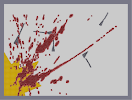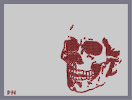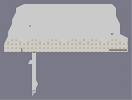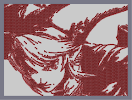Mined Your Own... Turtles' Ded Eternal Rest sea of urine Anime Twins Link

Pages: (2) [ 1 ] 2

### how do you

create such great n arts????

### agree with Bretzel..

Bloody Awesome! :D

5aved

AMAZING! 5aved

plz?

### woah

WOAH THAT IS SOOOO COOL YOUR N-ARTS ROCK MY SOCKS!

### Holy Shit

Holy F****ng S*** Your art is the best

### dude, i just had to rate this one.

i like dragons a lot.

### Perfect

That it's been rated 5 on average by exactly 100 people.

### OMFG

F*** this is awesome! 5aved

Voted.

### has anyone evr..

told you your great at n art?

DUH!!!!!!!!!!!!!!

how long this?

5AVED

### Awesome

5aved I'm gonna have to try this.

### WOw

nice
i used the same method to make http://nmaps.net/126646, which looks very similar to this. maybe they were based on the same picture...

Just amazing...

5/5

### \/\/0\/\/ translation: wow

|n(|2Ed|8|_e
translation: incredible

### niiice

kno strikes again with another great. love it. would give it 4.75 if i could but i cant so i round it off to the nearest whole = 5/5

amaazing

### I want to give it a 5

but I'm tired of N-arts hogging the top rated page. NR, but that doesn't mean I don't think it's an amazing piece of work.

amazing

### not 5 anymore.

you lost it somewhere in the 50s. but still the top map.

### 52

creative?
Don't even suggest it. Listen. If you don't dedicate it to me, don't expect me to be happy with it.
Ha, kidding.

Really, though, I did eat it.

and still a 5/5.

5/5

### Came across this

Im guessing thats the image you used

dang

### This

this this this ... is beautiful.

5/5

### 5/5 by 43 in a row

does that make this map like, the greatest ever?
41

### Oh my god...

Its my first time i see a map rated 5 O.O

Wow. Awesome, really.

5/5, ofcourse.
34

### ah k

nice, wait you ate my dragon???

@ barba, it's creative, i created something...
I also dedicated it to dragon moon for a reason, and I wanted to make him a dragon in my style...
also i dont have enough time to come up with completely fresh ideas let alone make art...
"do you mean 'turtles are dragons'?"

No. Back in the 14th century, when the dinosaurs ruled the land, and dragons blackened the skys, the turtles were the ones keeping population in order by eating dinosaurs. You're so silly!

### 33

I still haven't rated. :/

I agree with barba

### 32

and still has an average rating of 5. Phenomenal.